• 哈夫曼树/赫夫曼树 Python实现赫夫曼树 Python实现哈夫曼树 哈夫曼编码 哈夫曼压缩 哈夫曼解压 最简单的方式实现哈夫曼树
阅读目录基本概述构建赫夫曼树 思路Python实现构建赫夫曼树补充知识点：列表中如何按照元素的对象、类进行排序？实现 创建赫夫曼树赫夫曼编码数据压缩：创建赫夫曼树补充知识点：如何获取字符串的字节数？补充知识点：如何统计出字符串中每个字符的次数？补充知识点：如何给字典排序Python 实现数据压缩（创建赫夫曼树）赫夫曼编码 和 赫夫曼编码后数据补充知识点：如何遍历出二叉树的所有路径实现赫夫曼编码数据压缩：赫夫曼编码字节数组封装代码数据解压
基本概述

概念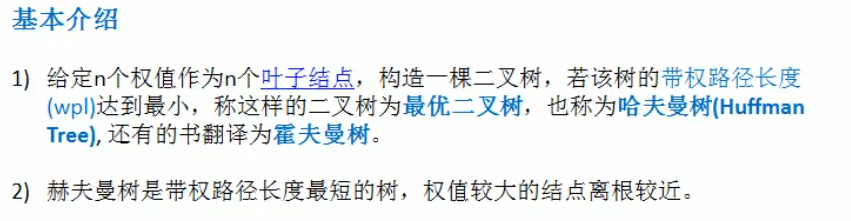解析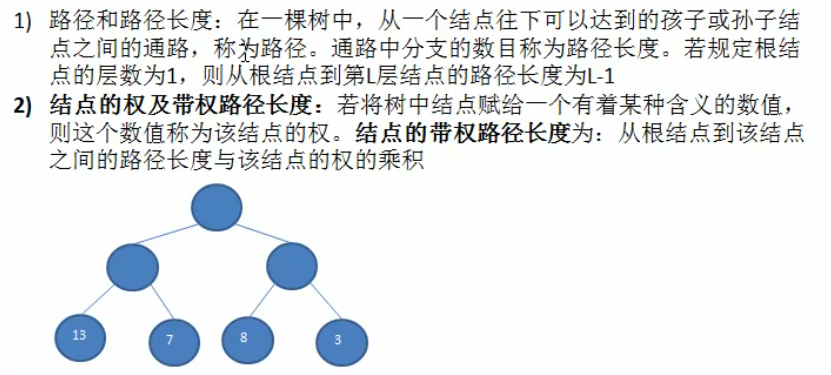举例，如上图中 权值为13 的结点，那么它的“结点带权路径长度”为：13*（3-1）=26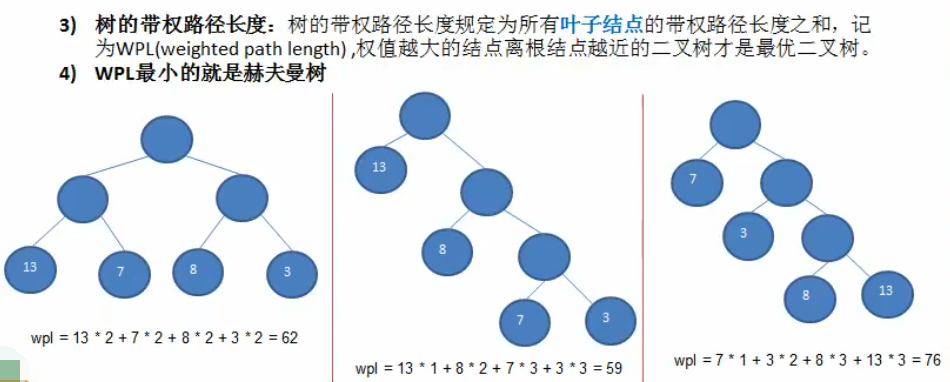上图中，中间的二叉树，wpl最小，而且权值为13的结点，满足“权值越大离根结点越近” 那么它就是赫夫曼树！
构建赫夫曼树 思路

构建赫夫曼树的步骤
以数列：[13,7,8,3,29,6,1 ] 为例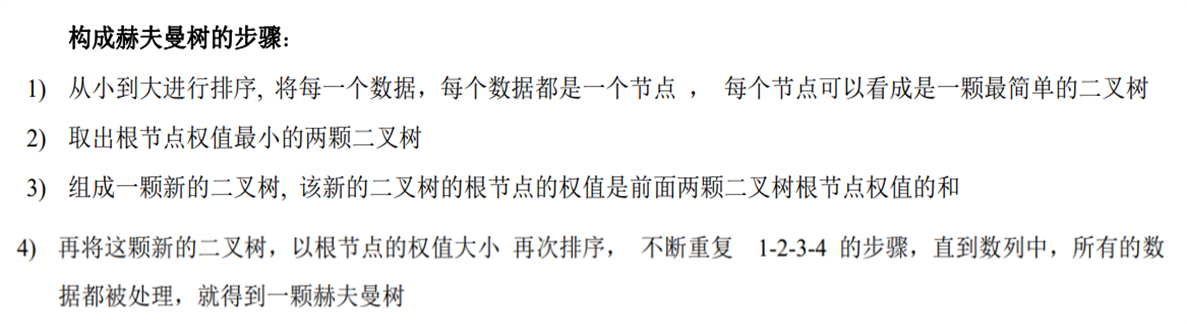注释：
（1）每一个结点可以看成一个二叉树，二叉树的分类中有说到
（2）取出排序后，前两个数；就是取出根结点权值最小的两颗二叉树

图解
（1）原数组 [13,7,8,3,29,6,1 ]，第一次升序后：[1,3,6,7,8,13,29]，取出前两个数（看下图的最底层），权值的和为新的根结点 4
（2）重新将数组连同刚刚产生的根结点4进行升序，数组为[4,6,7,8,13,29]，此时选出的前两个数是4和6（看下图中的倒数第二层），让它们权值的和为新的根结点 10，将10放入剩下的未处理数组中：[10,7,8,13,29]
（3）再次升序时，会发现 10 应该排在 7和8的后面，所以升序后的数组为：[7,8,10,13,29]，取出的前两个数是7和8，此时它们 应该和 根结点10 位于同一层，即倒数第三层，而且应该在10的前面（看下图的倒数第三层），因为它们是两个数 独立形成一个新的二叉树 那么在同一层的 [10,13,29] 前两个应该再生成一个新的根结点即是 23 （看下图倒数第四层）
（4）让7和8相加，形成新的根结点15 ，将15放入剩下的未处理数组中：[15,23,29]，以此类推，具体看下图，下图是从底层向上操作的↓↓↓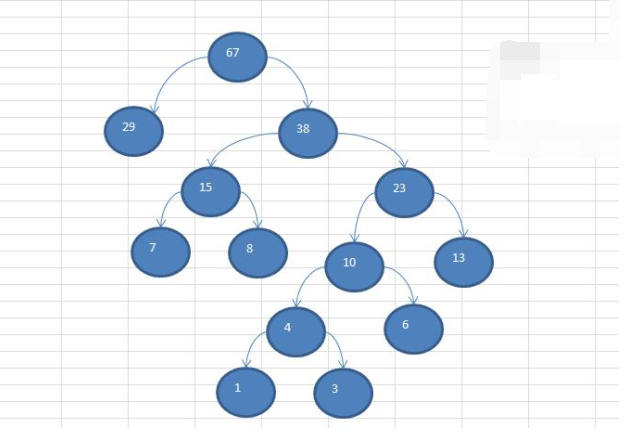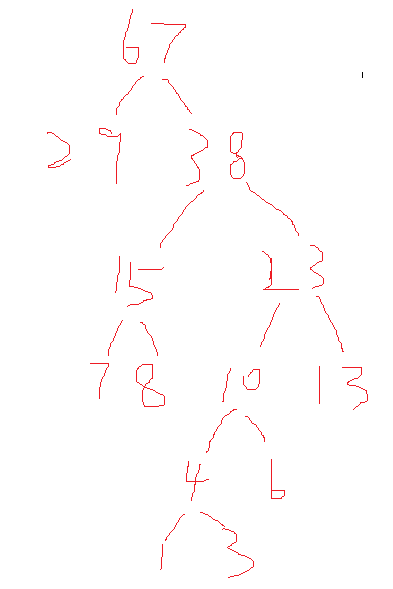Python实现构建赫夫曼树

先解决：每次从列表中选出最小的2个数
（1）第一种思路：遍历数组，进行比较；思路很好，但是没调用内置排序方法效率高

array = [4, 20, 3, 4, 5, 6, 7]
num1 = num2 = float('inf')
for x in range(0, len(array)):
if num1 > array[x]:
num2 = num1
num1 = array[x]
elif num2 > array[x]:
num2 = array[x]

print(num1, num2)

补充知识点：列表中如何按照元素的对象、类进行排序？

Java中通过实现 Comparable接口，可以给对象排序，Python中也可以在列表中按照元素的对象、类进行排序！
两种方法： operator模块中实现列表中的对象排序 和 如何用内置的sort()函数实现对象的排序

import operator  # 导入operator 包,pip install operator

lis = []  # 待排序列表
class Infor:  # 自定义的元素
def __init__(self, stu_id, name, age):
self.stu_id = stu_id
self.name = name
self.age = age

# 将对象加入列表中
lis.append(Infor(1, 'cbc', '11'))
lis.append(Infor(15, 'acd', '13'))
lis.append(Infor(6, 'bcd', '16'))
lis.append(Infor(6, 'acd', '18'))
lis.append(Infor(8, 'acd', '18'))

'''operater模块:适用于数据量大'''
# ----排序操作
# 参数为排序依据的属性，可以有多个，这里优先id，使用时按需求改换参数即可
temp = operator.attrgetter('stu_id', 'name')
lis.sort(key=temp)  # 使用时改变列表名即可
# ----排序操作
# 此时lis已经变成排好序的状态了，排序按照stu_id优先，其次是name，遍历输出查看结果
for obj in lis:
print(obj.stu_id, obj.name, obj.age)

'''内置的sort()'''
lis.sort(cmp=None, key=lambda x:x.stu_id, reverse=False)
# lis.sort(cmp=None, key=lambda x:(x.stu_id,x.name,...), reverse=False) # 多个指标，前主后次
for obj in lis:
print(obj.stu_id, obj.name, obj.age)

注意：这两种方式都不能对空值进行操作，如果有空值会报错：TypeError: ‘<’ not supported between instances of ‘NoneType’ and ‘int’
解决办法是：将空值换成“0”，这点相对于java实现 Comparable接口方式，有点不方便
实现 创建赫夫曼树
import operator

class TreeNode(object):
def __init__(self, val):
self.val = val
self.left = None
self.right = None

class HuffmanTree(object):
def create_huffman_tree(self, array):
'''
创建赫夫曼树的方法
:param array: 需要创建成赫夫曼树的数组
:return: 创建好后的赫夫曼树的root结点
'''
# 为了操作方便，先遍历array数组，将array的每个元素构成一个TreeNode
# 将TreeNode 对象放到 一个新的列表中
nodes = []
for item in array:
nodes.append(TreeNode(item))
# 对象排序
while len(nodes) > 1:  # 到最后只有个新的根结点就停止
temp = operator.attrgetter("val")
nodes.sort(key=temp)
# 取出根结点权值最小的两颗二叉树（结点）
# left_node = nodes
# right_node = nodes
# 可以直接用pop第一个元素，下面的remove就不用写了
left_node = nodes.pop(0)
right_node = nodes.pop(0)
# 构建一棵新的二叉树
parent_node = TreeNode(left_node.val + right_node.val)
parent_node.left = left_node  # 将父结点和左结点链接起来
parent_node.right = right_node
# 从nodes删除处理过的二叉树
# nodes.remove(left_node)
# nodes.remove(right_node)
# 将parent_node加入nodes
nodes.append(parent_node)
return nodes  # 返回根结点即可

def pre_order(self, node):  # node 为根结点，前序遍历
if node is None:
return
print(node.val, end=" ")
self.pre_order(node.left)
self.pre_order(node.right)

if __name__ == '__main__':
li = [13, 7, 8, 3, 29, 6, 1]
h = HuffmanTree()
huff_root = h.create_huffman_tree(li)
h.pre_order(huff_root)
'''输出结果：
67 29 38 15 7 8 23 10 4 1 3 6 13
'''


可以将上述结果，对照下图的前序遍历结果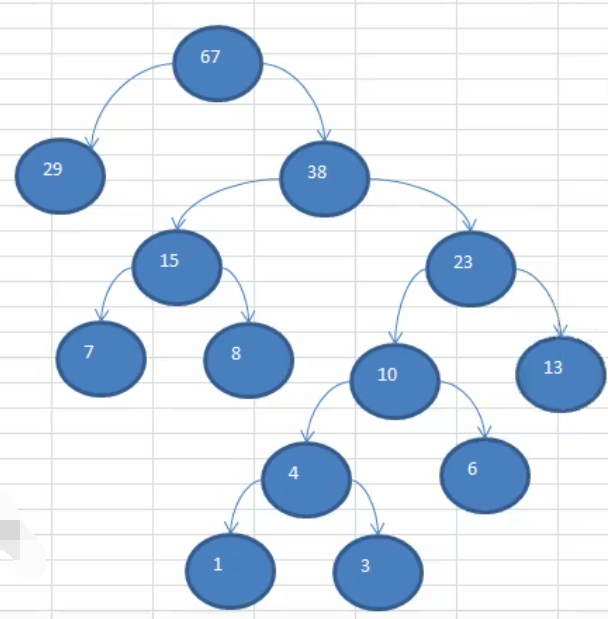赫夫曼编码

介绍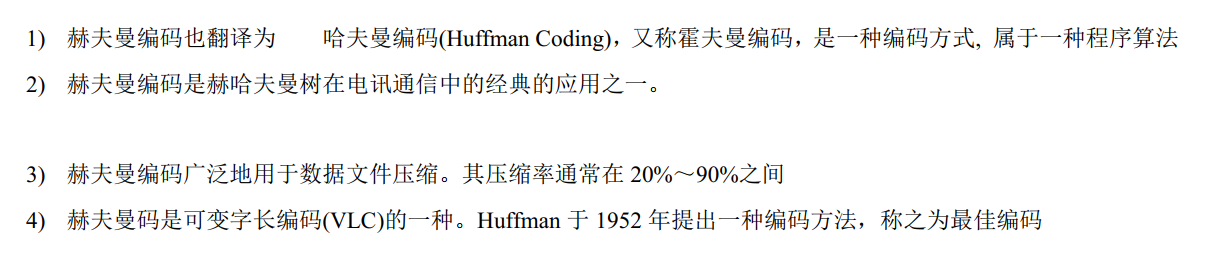原理剖析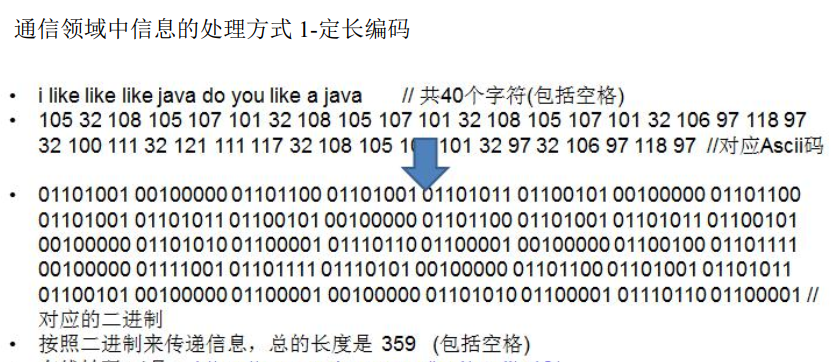变长编码：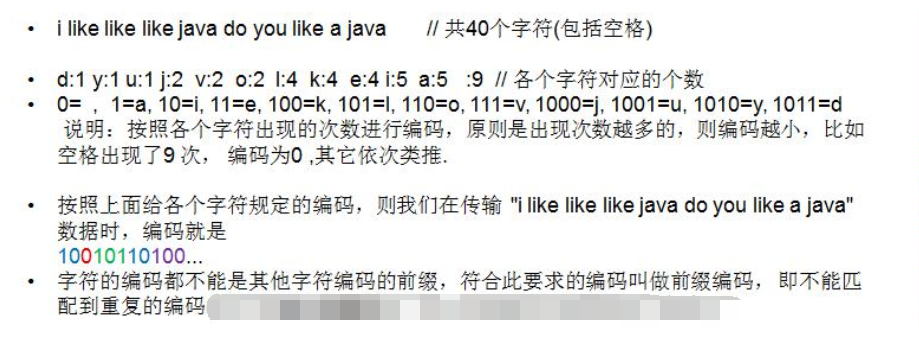赫夫曼编码 实现步骤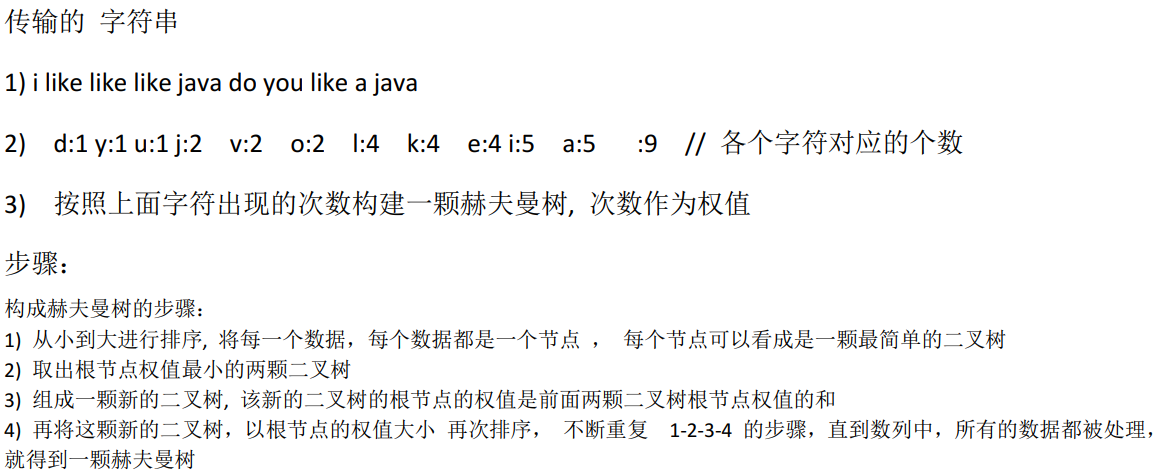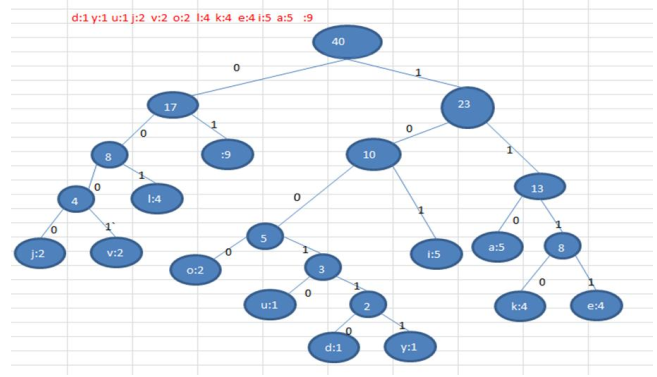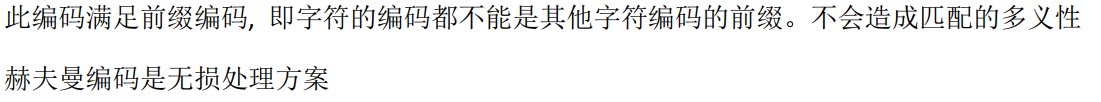赫夫曼编码生成的就是前缀编码：因为 统计的每个字符 都是赫夫曼树的叶子结点，路径都是唯一的，所以生成的编码是唯一的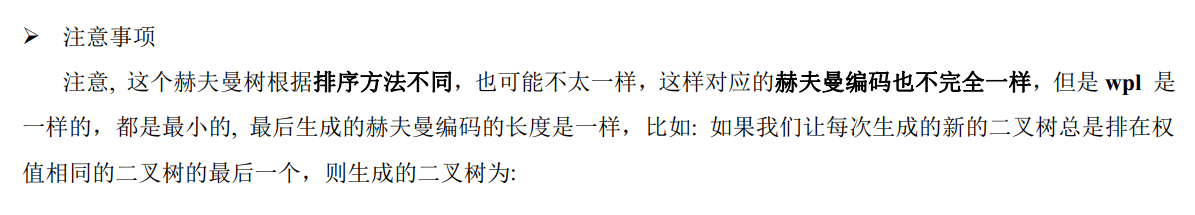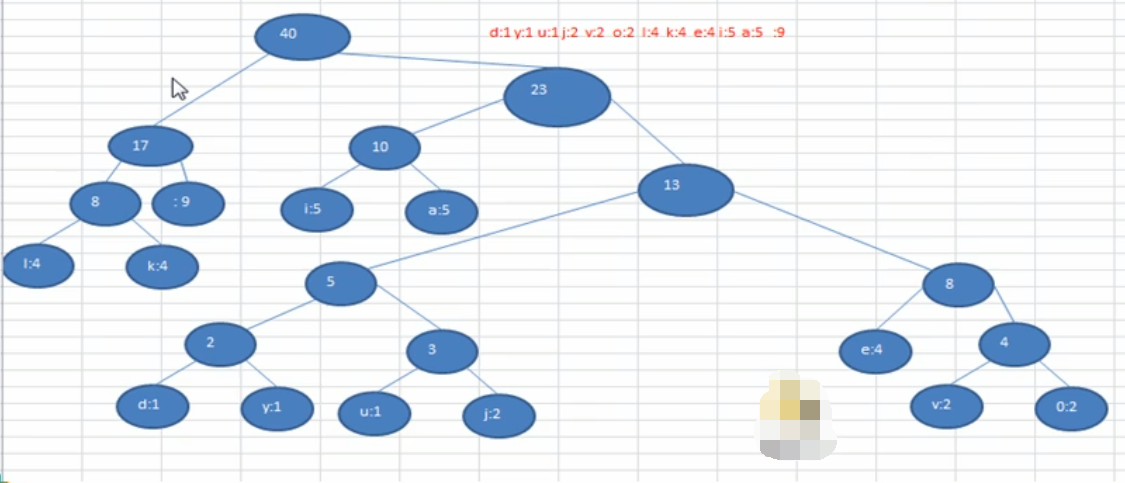数据压缩：创建赫夫曼树

步骤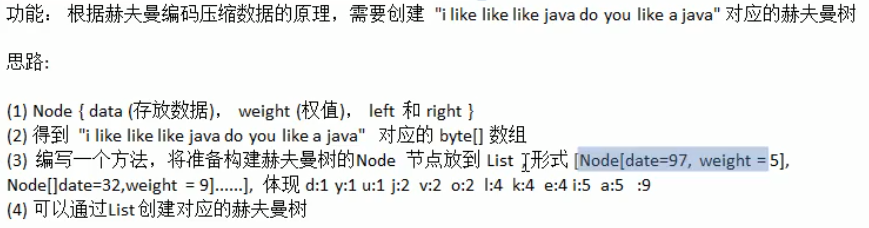Python 实现

补充知识点：如何获取字符串的字节数？
（1）java中可以先用 getBytes 然后.length 获取字符串的字节数，Python可以通过 len()函数获取字符串长度或字节数
（2）len 函数的基本语法格式为：len(string) ，它的结果是获取字符串的长度
（3）在 Python 中，不同的字符所占的字节数不同，数字、英文字母、小数点、下划线以及空格，各占一个字节，而一个汉字可能占 2~4 个字节，具体占多少个，取决于采用的编码方式。例如，汉字在 GBK/GB2312 编码中占用 2 个字节，而在 UTF-8 编码中一般占用 3 个字节。
以 UTF-8 编码为例，字符串“人生苦短，我用Python”所占用的字节数如下图所示。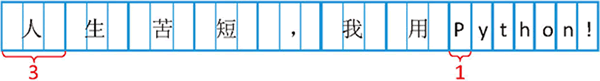可以通过使用 encode() 方法，将字符串进行编码后再获取它的字节数。例如，采用 UTF-8 编码方式，计算“人生苦短，我用Python”的字节数：
# utf-8
str1 = "人生苦短，我用Python"
print(len(str1.encode())) # 27
# 汉字加中文标点符号共 7 个，占 21 个字节，而英文字母和英文的标点符号占 6 个字节，
# 一共占用 27 个字节

# gbk
str1 = "人生苦短，我用Python"
print(len(str1.encode('gbk'))) # 20

补充知识点：如何统计出字符串中每个字符的次数？

方法一：直接使用字典

'''法一'''
s = 'helloworld'
d = dict()
for x in s:
if x not in d:
d[x] = 1
else:
d[x] = d[x] + 1
print(d) # {'h': 1, 'e': 1, 'l': 3, 'o': 2, 'w': 1, 'r': 1, 'd': 1}

'''法二'''
s = 'helloworld'
d2 = dict()
for x in s:
d2[x] = d2.get(x, 0) + 1
print(d2) # {'h': 1, 'e': 1, 'l': 3, 'o': 2, 'w': 1, 'r': 1, 'd': 1}

'''法三：字符串count结合使用'''
s = 'helloworld'
d3 = dict()
for x in s:
d3[x] = s.count(x)
print(d3) # {'h': 1, 'e': 1, 'l': 3, 'o': 2, 'w': 1, 'r': 1, 'd': 1}

# 至于先用set()集合去重，然后遍历原字符串用count统计出现次数的方法，类似就不加赘述了


方式二：制表

def count_char(str):
str = str.lower()  # 化成小写
ans = []
for i in range(26):  # 列表赋初值  26 个 0
ans.append(0)
for i in str:
if (ord(i) >= ord('a') and ord(i) <= ord('z')):
ans[ord(i) - ord('a')] = ans[ord(i) - ord('a')] + 1  # 统计个数
return ans

if __name__ == "__main__":
str = input()
print(count_char(str))

#-------
def count_char(st):  # 定义数个数的函数
keys = [chr(i + 97) for i in range(26)]  # 生成26个字母的key列表
di = dict().fromkeys(keys, 0)  # 赋给每个key初始值0
new = []  # 建立一个新列表用于存放有序的key
st = st.lower()  # 将所有输入的字符改为小写
for s in st:  # 遍历字符串
di[s] = st.count(s)  # 输出每个字符的个数，存放到字典里
for k in keys:  # 遍历keys,将其在di中的值添加到新列表，获得有序的26个字母的个数
new.append(di[k])
return new  # 返回存有26个字母个数的列表

if __name__ == "__main__":
st = input()  # 输入字符串
str1 = ""  # 定义一个空字符串
for s in st:  # 遍历输入的字符串
if s.isalpha() != 0:  # 只有字母才添加到新字符串，标点忽略不计
str1 += s
print(count_char(str1))  # 输出列表


方法三：Counter（计数器）：用于追踪值的出现次数；Counter类继承dict类，所以它能使用dict类里面的方法

特别注意： 它的统计输出是无序的
'''直接导入类用法'''
from collections import Counter

str_exp = ' aaffbbcccdddee '
c = Counter()  # 先新建实例
for ele in str_exp:
c[ele] = c[ele] + 1
print(c, type(c)) # Counter({'c': 3, 'd': 3, ' ': 2, 'a': 2, 'f': 2, 'b': 2, 'e': 2})
# 注意c的类型不是字典：<class 'collections.Counter'>
# 继承dict类中的 items()方法
for key, value in c.items():
print(key, value, end=" | ") #  2 | a 2 | f 2 | b 2 | c 3 | d 3 | e 2 |


'''导入collections模块'''
import collections
obj = collections.Counter('aabbccc')
print(obj)
# 输出：Counter({'c': 3, 'a': 2, 'b': 2}) 它的类型不是字典是<class 'collections.Counter'

'''elements()方法'''
import collections
obj = collections.Counter('aabbccc')
print(sorted(obj.elements()))
#输出：['a', 'a', 'b', 'b', 'c', 'c', 'c']
for k in obj.elements():   #遍历打印obj所有元素
print(k)

'''most_common(指定一个参数n，列出前n个元素，不指定参数，则列出所有)'''
import collections
obj = collections.Counter('aabbbcccc')
print(obj.most_common(2))
#输出：[('c', 4), ('b', 3)]

'''items(从dict类中继承的方法)'''
import collections
obj = collections.Counter('aabbbcccc')
print(obj.items())

for k,v in obj.items():
print(k,v)

# 输出：dict_items([('b', 3), ('c', 4), ('a', 2)])
#     b 3
#     c 4
#     a 2

'''update(增加元素)'''
import collections
obj = collections.Counter(['11','22'])
obj.update(['22','55'])
print(obj)

#输出：Counter({'22': 2, '11': 1, '55': 1})

'''subtract(原来的元素减去新传入的元素)'''
import collections
obj = collections.Counter(['11','22','33'])
obj.subtract(['22','55'])
print(obj)

#输出：Counter({'11': 1, '33': 1, '22': 0, '55': -1})

补充知识点：如何给字典排序

说明：给字典排序 是为了让最后创建的结果更贴近所参考的教程，总体wpl是一样的，所以排不排序都无所谓；因为java在使用map[key,value] 在统计字符串出现的次数时，会自动按照 key 的大小升序排列！
字典排序的方法，字典用法的总结：字典排序

Python 实现数据压缩（创建赫夫曼树）
import operator
from collections import Counter
from operator import itemgetter

class TreeNode(object):
def __init__(self, val, weight):
self.val = val  # 存放数据（字符）本身，如"a" 或者"97"
self.weight = weight  # 存放出现的次数
self.left = None
self.right = None

class HuffmanCode(object):
def get_nodes(self, bytes_array):

nodes = []
# 存储每个byte出现的次数
# 统计一个字符串中每个元素出现的次数有很多种，这里为了操作方便，用Counter
'''法一
c = Counter()  # 创建一个统计对象
for item in bytes_array:
c[item] = c[item] + 1
# 此时 c 会用字典的形式保存：key:每个元素；value：出现的次数
'''
# 法二 使用字典
dic = {}
for i in bytes_array:
if i not in dic:
dic[i] = 1
else:
dic[i] += 1
'''如果不排序
for key, value in dic.items():
nodes.append(TreeNode(key, value))
'''

'''第一种排序 键值对 方式：
dic_list = sorted(dic.items(), key=lambda x: x)
print(dic_list)
for index, value in enumerate(dic_list):  # 拿到 每一组键值对
nodes.append(TreeNode(value, value))  # 创建结点对象
'''

# 第二种排序 键值对 方式：
dic_list = sorted(dic.items(), key=itemgetter(0), reverse=False)
for item in dic_list:
nodes.append(TreeNode(item, item))

return nodes

def create_huffman_tree(self, nodes):
while len(nodes) > 1:
# 法一：nodes.sort(key=lambda x: x.weight)
# 法二
nodes.sort(key=operator.attrgetter("weight"))
# 取出根结点权值最小的两颗二叉树（结点）
left_node = nodes.pop(0)
right_node = nodes.pop(0)
# 构建一棵新的二叉树,它的根结点（不是叶子结点）没有val，用0补充；含有weight
parent_node = TreeNode(0, left_node.weight + right_node.weight)
parent_node.left = left_node  # 将父结点和左结点链接起来
parent_node.right = right_node
# 将parent_node加入nodes
nodes.append(parent_node)
return nodes  # 返回根结点即可

def pre_order(self, node):  # 前序遍历 传入node为根结点
if node is None:
return
print(node.val, node.weight, end="  || ")
self.pre_order(node.left)
self.pre_order(node.right)

if __name__ == '__main__':
content = "i like like like java do you like a java"
# content_bytes = list(content.encode('utf-8'))
content_bytes = content.encode('utf-8')
print(len(content_bytes))
h = HuffmanCode()
# 获取到结点列表
obj_list = h.get_nodes(content_bytes)
# 获取到赫夫曼树的根结点
huffman_root = h.create_huffman_tree(obj_list)
# 前序遍历
h.pre_order(huffman_root)

'''
40

[(32, 9), (97, 5), (100, 1), (101, 4), (105, 5), (106, 2), (107, 4), (108, 4), (111, 2), (117, 1), (118, 2), (121, 1)] # 只是给字典排了下序

0 40  || 0 17  || 0 8  || 108 4  || 0 4  || 111 2  || 118 2  || 32 9  || 0 23  || 0 10  || 97 5  || 105 5  || 0 13  || 0 5  || 0 2  || 100 1  || 117 1  || 0 3  || 121 1  || 106 2  || 0 8  || 101 4  || 107 4  ||
# 赫夫曼树在处理weight一样时，是按照排序规则来合并结点，所以形态会不一样，但是最后根结点是一样的，
所以 看val为空的结点，间隔不管形态如何，间隔一定会一样的
'''

赫夫曼编码 和 赫夫曼编码后数据

到这一步，我们要做的是编码，因为所有的val有值得结点，必然是位于赫夫曼树的叶子节点，按照上面的规定，从根结点出发，遍历每一条到叶子结点的路径，走过的路径向左则为0，向右则为1，因为之前学过遍历二叉树路径的方法，所以可以这样很巧妙的完成！

补充知识点：如何遍历出二叉树的所有路径

如下图有一颗10个结点的二叉树：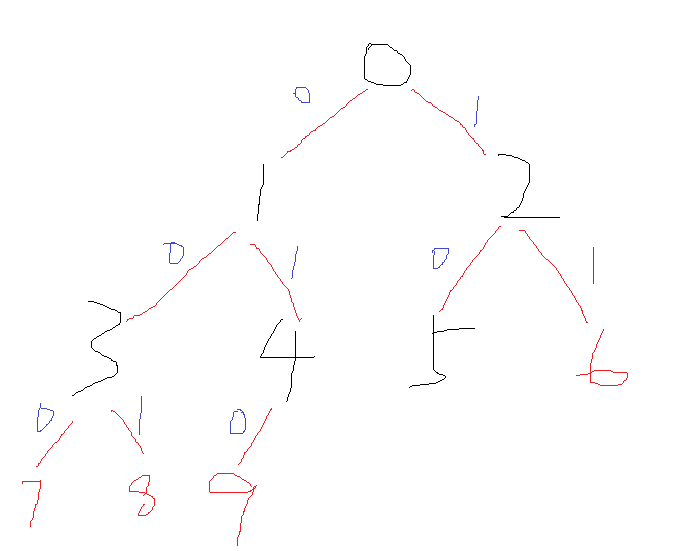该怎么求它的所有路径？我们可以这样做
（1）在什么情况下输出结点？假设只有一个根结点root，它没有左右子结点，此时就输出结点的值，所以如上图的二叉树，我们使用递归，找到每一个没有左右子结点的结点，如上图是：[7,8,9,5,6]，所以我们的递归退出条件找到了，即是：存在当前结点并且它没有左右子结点了，那么就退出
（2）为了串联起走过的路径，我们需要新增一个全局变量path，每次将走过的结点以字符串的形式串联起来，新增一个结果列表，输出最后结果！

class TreeNote(object):  # 创建树的结点
def __init__(self, val=-1):
self.val = val
self.left = None
self.right = None

class BinaryTree(object):  # 创建二叉树
def __init__(self):
self.root = None  # 根结点

node = TreeNote(val)
if self.root is None:
self.root = node
return
queue = [self.root]
while queue:
temp_node = queue.pop(0)
if temp_node.left is None:
temp_node.left = node
return
else:
queue.append(temp_node.left)
if temp_node.right is None:
temp_node.right = node
return
else:
queue.append(temp_node.right)

def bre_order(self, node):  # 二叉树广度遍历
if node is None:
return
queue = [node]
while queue:
temp_node = queue.pop(0)
print(temp_node.val, end=" ")
if temp_node.left is not None:
queue.append(temp_node.left)
if temp_node.right is not None:
queue.append(temp_node.right)

def find_rode(self, node, path, res):  # node:传入root；path:路径；res：结果
if node is None:
return []
if node.left is None and node.right is None: # 当前结点没有左右子结点
res.append(path + str(node.val))
return res
if node.left: # 向左递归
self.find_rode(node.left, path + str(node.val), res)
if node.right: # 向右递归
self.find_rode(node.right, path + str(node.val), res)

if __name__ == '__main__':
t = BinaryTree()
for i in range(10):
print("\n广度遍历为:")
t.bre_order(t.root)
print()
out_put = []
t.find_rode(t.root, "", out_put)
print("二叉树所有路径为:")
print(out_put)
'''
广度遍历为:
0 1 2 3 4 5 6 7 8 9
二叉树所有路径为:
['0137', '0138', '0149', '025', '026']
'''

实现赫夫曼编码

知道了怎么求二叉树的所有路径，那么就可以轻松求出赫夫曼树所有路径，按照新规定：走过的路径我们只需要拿到叶子结点的 val 即可，其他结点不需要拿val，路径则按照左边以“0”替换，右边以“1”替换，即可！

import operator
from operator import itemgetter

class TreeNode(object):
def __init__(self, val, weight):
self.val = val  # 存放数据（字符）本身，如"a" 或者"97"
self.weight = weight  # 存放出现的次数
self.left = None
self.right = None

class HuffmanCode(object):
# 返回赫夫曼树的结点列表
def get_nodes(self, bytes_array):
nodes = []
dic = {}
for i in bytes_array:
if i not in dic:
dic[i] = 1
else:
dic[i] += 1
# 第二种排序 键值对 方式：
dic_list = sorted(dic.items(), key=itemgetter(0), reverse=False)
for item in dic_list:
nodes.append(TreeNode(item, item))

return nodes

# 构建赫夫曼树，返回赫夫曼树的根结点
def create_huffman_tree(self, nodes):
while len(nodes) > 1:
nodes.sort(key=operator.attrgetter("weight"))
# 取出根结点权值最小的两颗二叉树（结点）
left_node = nodes.pop(0)
right_node = nodes.pop(0)
# 构建一棵新的二叉树,它的根结点，没有val，只有weight
parent_node = TreeNode(0, left_node.weight + right_node.weight)
parent_node.left = left_node  # 将父结点和左结点链接起来
parent_node.right = right_node
# 将parent_node加入nodes
nodes.append(parent_node)
return nodes  # 返回根结点即可

# 为了更好比对图，我们可以使用 广度遍历测试
def bre_order(self, node):
if node is None:
return
queue = [node]
while queue:
temp_node = queue.pop(0)
print(temp_node.val, temp_node.weight, end=" || ")
if temp_node.left:
queue.append(temp_node.left)
if temp_node.right:
queue.append(temp_node.right)

# 获取赫夫曼编码表
def get_codes(self, node, path, temp):
if node is None:
return
if node.left is None and node.right is None:
temp.append(path + "|" + str(node.val)) # 中间新增一个分割符号，最后更好拿到编码和val
return temp
if node.left:
self.get_codes(node.left, path + "0", temp) # 左边让路径不断加上“0”
if node.right:
self.get_codes(node.right, path + "1", temp) # 左边让路径不断加上“1”

if __name__ == '__main__':
content = "i like like like java do you like a java"
content_bytes = content.encode('utf-8')
print(len(content_bytes))
h = HuffmanCode()
# 获取到结点列表
obj_list = h.get_nodes(content_bytes)
# 获取到赫夫曼树的根结点
huffman_root = h.create_huffman_tree(obj_list)
h.bre_order(huffman_root)
print()
# 赫夫曼编码
out_put = []
h.get_codes(huffman_root, "", out_put)
for item in out_put:
print(chr(int(item.split("|"))), item.split("|"), end=";")
print()
'''输出结果为
40
0 40 || 0 17 || 0 23 || 0 8 || 32 9 || 0 10 || 0 13 || 108 4 || 0 4 || 97 5 || 105 5 || 0 5 || 0 8 || 111 2 || 118 2 || 0 2 || 0 3 || 101 4 || 107 4 || 100 1 || 117 1 || 121 1 || 106 2 ||

val对应的编码为：
l 000;
o 0010;
v 0011;
01;
a 100;
i 101;
d 11000;
u 11001;
y 11010;
j 11011;
e 1110;
k 1111;
'''


验证一下是否正确：因为得到的赫夫曼树形态有可能不一样，得到的编码也不一样，但是按照上面写的，最后将上面每个val对应的新编码值，去组合一下输入的字符串，得到的字符长度要为：133

# 验证：手动输入（下一节实现自动生成如下编码，并且按照每八位压缩）
final_str = "1010100010100111110010001010011111001000101001111100111011100001110001110000010011101000101100101000101001111100110001110111000011100"
print(len(final_str)) # 133 恭喜我们做对了！

数据压缩：赫夫曼编码字节数组

按照上述步骤我们已经得到了每一个val的赫夫曼编码，并且存放在了数组中，接下来要做的就是自动将数组里的值生成上面的验证的：final_str 并且按8位 生成 赫夫曼编码字节数组
对于上面我们用 out_put = [ ] 列表来保存数据，我们可以改成，在生成赫夫曼编码时直接用字典来保存每条路径，即是：dict[node.val]=path 这样在处理自动将输入的字符串转成赫夫曼编码，可以充分利用字典的特性，所以下面整体代码为：

import operator
from operator import itemgetter

class TreeNode(object):
def __init__(self, val, weight):
self.val = val  # 存放数据（字符）本身，如"a" 或者"97"
self.weight = weight  # 存放出现的次数
self.left = None
self.right = None

class HuffmanCode(object):
# 返回赫夫曼树的结点列表
def get_nodes(self, bytes_array):
nodes = []
dic = {}
for i in bytes_array:
if i not in dic:
dic[i] = 1
else:
dic[i] += 1
# 第二种排序 键值对 方式：
dic_list = sorted(dic.items(), key=itemgetter(0), reverse=False)
for item in dic_list:
nodes.append(TreeNode(item, item))

return nodes

# 构建赫夫曼树，返回赫夫曼树的根结点
def create_huffman_tree(self, nodes):
while len(nodes) > 1:
nodes.sort(key=operator.attrgetter("weight"))
# 取出根结点权值最小的两颗二叉树（结点）
left_node = nodes.pop(0)
right_node = nodes.pop(0)
# 构建一棵新的二叉树,它的根结点，没有val，只有weight
parent_node = TreeNode(0, left_node.weight + right_node.weight)
parent_node.left = left_node  # 将父结点和左结点链接起来
parent_node.right = right_node
# 将parent_node加入nodes
nodes.append(parent_node)
return nodes  # 返回根结点即可

# 为了更好比对图，我们可以使用 广度遍历测试
def bre_order(self, node):
if node is None:
return
queue = [node]
while queue:
temp_node = queue.pop(0)
print(temp_node.val, temp_node.weight, end=" || ")
if temp_node.left:
queue.append(temp_node.left)
if temp_node.right:
queue.append(temp_node.right)

# 获取赫夫曼编码（每一条路径）
def get_codes(self, node, path, temp):
if node is None:
return
if node.left is None and node.right is None:
temp[str(node.val)] = path # 改成字典来保存路径
return temp
if node.left:
self.get_codes(node.left, path + "0", temp) # 左边让路径不断加上“0”
if node.right:
self.get_codes(node.right, path + "1", temp) # 左边让路径不断加上“1”

def zip_codes(self, codes_array, srt_byte_content, str_join): # 充分利用字典特性
# 参数：传入赫夫曼编码数组，传入一个字节字符串，拼接字符串
for item in srt_byte_content: # 遍历每一个字符串字节
str_join += str(codes_array.get(str(item))) # 通过字节作为key，去找它的值，再拼接
return str_join

if __name__ == '__main__':
content = "i like like like java do you like a java"
content_bytes = content.encode('utf-8')
# print(len(content_bytes))
h = HuffmanCode()
# 获取到结点列表
obj_list = h.get_nodes(content_bytes)
# 获取到赫夫曼树的根结点
huffman_root = h.create_huffman_tree(obj_list)
# 广度遍历
# h.bre_order(huffman_root)
# 编码后
out_put = {} # 改成字典
h.get_codes(huffman_root, "", out_put)
str_join = " "
final_str = h.zip_codes(out_put, content_bytes, str_join)
print(final_str)
''' 输出结果
1010100010111111110010001011111111001000101111111100111011100001110001110000010011101000
101100101000101111111100110001110111000011100
'''


接下来需要将自动获取到的字符串 转成 byte数组
（1）先要创建一个存下字符串的数组，有两种方式计算需要的数组长度，但是Python不需要规定长度也行，直接append添加每8个一组，最后多少都无所谓，emmmm!!!

第一种写法：

if len(str_join) % 8 == 0: # 自动获取的字符串长度先模上8
byte_array_len = len(str_join) / 8
else:
byte_array_len = len(str_join) // 8 + 1
byte_array =  * byte_array_len
print(len(byte_array)) # 17


第二种写法：

byte_array_len = (len(str_join) + 7) // 8
byte_array =  * byte_array_len
print(len(byte_array)) # 17


实现赫夫曼编码 转成 byte数组

'''上面代码一模一样就不拷贝了'''
def zip_codes(self, codes_array, srt_byte_content, str_join):  # 参数：传入一个字节字符串，传入赫夫曼编码数组
for item in srt_byte_content:
str_join += str(codes_array.get(str(item)))
byte_array = []
final_byte_array = []
for i in range(1, len(str_join), 8):  # 步长取8,从第二位开始，第一位是个空格
byte_array.append(str_join[i:i + 8])
for item in byte_array:
print（item） # 输出检查
if item == "1":
final_byte_array.append(int(item, base=2) - 256)  # 说明一
else:
final_byte_array.append(int(item, base=2))
return final_byte_array

if __name__ == '__main__':
content = "i like like like java do you like a java"
content_bytes = content.encode('utf-8')
# print(len(content_bytes))
h = HuffmanCode()
# 获取到结点列表
obj_list = h.get_nodes(content_bytes)
# 获取到赫夫曼树的根结点
huffman_root = h.create_huffman_tree(obj_list)
# 前序遍历
# h.pre_order(huffman_root)
# print()
# h.bre_order(huffman_root)
# print()
# 编码后
out_put = {}
h.get_codes(huffman_root, "", out_put)
str_join = " "
# final_str = h.zip_codes(out_put, content_bytes, str_join)
final_byte = h.zip_codes(out_put, content_bytes, str_join)
print(final_byte)
print(len(final_byte)

'''输出结果
10101000
10111111
11001000
10111111
11001000
10111111
11001110
11100001
11000111
00000100
11101000
10110010
10001011
11111100
11000111
01110000
11100

[-88, -65, -56, -65, -56, -65, -50, -31, -57, 4, -24, -78, -117, -4, -57, 112, -228]

17
---
可以计算压缩率为：（40-17）/ 40 = 0.575
'''


说明一：默认切割出来的每八位，在计算机底层应该将它当做补码 才能进行存储，所以符号位为1的二进制码，表明它的原码是一个负数，那么怎么通过补码求出该数的十进制数？可以用快捷方法：算出补码无视符号位在二进制中的数 减去 256 即可！可以自行通过每个切割出来的字符串，当做补码然后求出它的原码，去掉符号位，求出二进制数，来验证！

封装代码

为了调用方便，我们将代码都封装到一个方法里，然后主函数调用该方法执行，主函数声明的全局变量，也都放入到一个类中，于是代码可以精简为：↓↓↓

import operator
from operator import itemgetter

class TreeNode(object):
def __init__(self, val, weight):
self.val = val  # 存放数据（字符）本身，如"a" 或者"97"
self.weight = weight  # 存放出现的次数
self.left = None
self.right = None

class HuffmanCode(object):
out_put_dic = {}  # 键值对：字符字节：赫夫曼编码
str_join = ""  # 拼接字符串

# 返回赫夫曼树的结点列表
def get_nodes(self, bytes_array):
nodes = []
dic = {}
for i in bytes_array:
if i not in dic:
dic[i] = 1
else:
dic[i] += 1
# 给键值对 排序（可以有可无）
dic_list = sorted(dic.items(), key=itemgetter(0), reverse=False)
for item in dic_list:
nodes.append(TreeNode(item, item))
return nodes

# 构建赫夫曼树，返回赫夫曼树的根结点
def create_huffman_tree(self, nodes):
while len(nodes) > 1:
nodes.sort(key=operator.attrgetter("weight"))
# 取出根结点权值最小的两颗二叉树（结点）
left_node = nodes.pop(0)
right_node = nodes.pop(0)
# 构建一棵新的二叉树,它的根结点，没有val，只有weight
parent_node = TreeNode(0, left_node.weight + right_node.weight)
parent_node.left = left_node  # 将父结点和左结点链接起来
parent_node.right = right_node
# 将parent_node加入nodes
nodes.append(parent_node)
return nodes  # 返回根结点即可

# 获取赫夫曼编码表
def get_codes(self, node, path, temp):
if node is None:
return
if node.left is None and node.right is None:
temp[str(node.val)] = path
return temp
if node.left:
self.get_codes(node.left, path + "0", temp)
if node.right:
self.get_codes(node.right, path + "1", temp)
return temp

def zip_codes(self, codes_array, srt_content_byte, str_join):  # 参数：传入一个字节字符串，传入赫夫曼编码数组
for item in srt_content_byte:
str_join += str(codes_array.get(str(item)))
byte_array = []
final_byte_array = []
for i in range(0, len(str_join), 8):  # 步长取8,从第二位开始，第一位是个空格
byte_array.append(str_join[i:i + 8])
for item in byte_array:
if item == "1":
final_byte_array.append(int(item, base=2) - 256)
else:
final_byte_array.append(int(item, base=2))
return final_byte_array

def huffman_zip(self, bytes_array):
'''
封装
:param bytes_array: 传入一个原始字节数组
:return: 返回一个压缩字节数组
'''
nodes = self.get_nodes(bytes_array)
huffman_tree_root = self.create_huffman_tree(nodes)
codes_array = self.get_codes(huffman_tree_root, "", HuffmanCode.out_put_dic)
get_zip_byte = self.zip_codes(codes_array, bytes_array, HuffmanCode.str_join)
return get_zip_byte

if __name__ == '__main__':
content = "i like like like java do you like a java"
content_bytes = content.encode('utf-8')
h = HuffmanCode()
final = h.huffman_zip(content_bytes)
print(final)
'''输出结果
[-88, -65, -56, -65, -56, -65, -50, -31, -57, 4, -24, -78, -117, -4, -57, 112, -228]
'''

数据解压

第一步是还原回原来的赫夫曼编码字符串：即是通过最后的压缩字节数组，得到：“1010100010111111110010001011111111001000101111111100111011100001110001110000010011101000101100101000101111111100110001110111000011100” 我们可以遍历数组，如果是负数先加回265，如果是正数就不要加，但是这时候要注意两个重要问题：
（1）如果压缩字节数组还没遍历到最后一个数，遇到正数，在正数转成二进制码时，需要补齐八位，即前面要补上0凑足八位二进制；
（2）最后一位，如果是负数直接拼接起二进制码，如果是正数，不要补齐八位；因为前面我们在切割字符串时，是按照8位来切割的，最后剩下的，是直接转二进制的

'''前面代码一样，就省略下了'''
def be_back_str(self, huffman_array):  # 调用后,返回拼接哈夫曼编码
# for ele in huffman_array: # 因为最后一个是负数，所以直接拼接上了，但是也可能是正数
#     if ele < 0:
#         HuffmanCode.str_join += str(bin(ele + 256))[2:]
#     else:
#         add_zero = 8 - len(bin(ele)[2:])
#         HuffmanCode.str_join += ("0" * add_zero + bin(ele)[2:])
# print(HuffmanCode.str_join)
for i in range(len(huffman_array) - 1): # 遍历到数组前一个
if huffman_array[i] < 0:
HuffmanCode.str_join += str(bin(huffman_array[i] + 256))[2:]
else:
HuffmanCode.str_join += ("0" * add_zero + bin(huffman_array[i])[2:])
if huffman_array[-1] < 0: # 考虑最后一个数的两种情况
HuffmanCode.str_join += bin(huffman_array[-1] + 256)[2:]
else:
HuffmanCode.str_join += bin(huffman_array[-1])[2:]
print(HuffmanCode.str_join) # 打印测试下

if __name__ == '__main__':
content = "i like like like java do you like a java"
content_bytes = content.encode('utf-8')
h = HuffmanCode()
final = h.huffman_zip(content_bytes)
print(final)
h.be_back_str(final)
'''输出结果：
101010001011111111001000101111111100100010111111110011101110000111000111
0000010011101000101100101000101111111100110001110111000011100
比较和前面的一致
'''


第二步，查找字符串，然后去原来的字典查值，得到字节数组，最后返回原来的字符串；这里要用到字符串的查找！

完整代码

import operator
from operator import itemgetter

class TreeNode(object):
def __init__(self, val, weight):
self.val = val  # 存放数据（字符）本身，如"a" 或者"97"
self.weight = weight  # 存放出现的次数
self.left = None
self.right = None

class HuffmanCode(object):
out_put_dic = {}  # 键值对：字符字节：赫夫曼编码
str_join = ""  # 拼接字符串
final_bytes = []

# 返回赫夫曼树的结点列表
def get_nodes(self, bytes_array):
nodes = []
dic = {}
for i in bytes_array:
if i not in dic:
dic[i] = 1
else:
dic[i] += 1
# 给键值对 排序（可以有可无）
dic_list = sorted(dic.items(), key=itemgetter(0), reverse=False)
for item in dic_list:
nodes.append(TreeNode(item, item))
return nodes

# 构建赫夫曼树，返回赫夫曼树的根结点
def create_huffman_tree(self, nodes):
while len(nodes) > 1:
nodes.sort(key=operator.attrgetter("weight"))
# 取出根结点权值最小的两颗二叉树（结点）
left_node = nodes.pop(0)
right_node = nodes.pop(0)
# 构建一棵新的二叉树,它的根结点，没有val，只有weight
parent_node = TreeNode(0, left_node.weight + right_node.weight)
parent_node.left = left_node  # 将父结点和左结点链接起来
parent_node.right = right_node
# 将parent_node加入nodes
nodes.append(parent_node)
return nodes  # 返回根结点即可

# 获取赫夫曼编码表
def get_codes(self, node, path, temp):
if node is None:
return
if node.left is None and node.right is None:
temp[str(node.val)] = path
return temp
if node.left:
self.get_codes(node.left, path + "0", temp)
if node.right:
self.get_codes(node.right, path + "1", temp)
return temp

def zip_codes(self, codes_array, srt_content_byte, str_join):  # 参数：传入一个字节字符串，传入赫夫曼编码数组
for item in srt_content_byte:
str_join += str(codes_array.get(str(item)))
byte_array = []
final_byte_array = []
for i in range(0, len(str_join), 8):  # 步长取8,从第二位开始，第一位是个空格
byte_array.append(str_join[i:i + 8])
for item in byte_array:
if item == "1":
final_byte_array.append(int(item, base=2) - 256)
else:
final_byte_array.append(int(item, base=2))
return final_byte_array

def huffman_zip(self, bytes_array):
'''
封装
:param bytes_array: 传入一个原始字节数组
:return: 返回一个压缩字节数组
'''
nodes = self.get_nodes(bytes_array)
huffman_tree_root = self.create_huffman_tree(nodes)
codes_array = self.get_codes(huffman_tree_root, "", HuffmanCode.out_put_dic)
get_zip_byte = self.zip_codes(codes_array, content_bytes, HuffmanCode.str_join)
return codes_array, get_zip_byte

def be_back_str(self, huffman_array, codes_dict):
'''
:param huffman_array: 最后返回的哈夫曼编码字节数组
:param codes_dict: 最开始存放字节对应的编码，键值对为>>>字节：哈夫曼编码
:return: 返回原来的字符串
'''
for i in range(len(huffman_array) - 1):
if huffman_array[i] < 0:
HuffmanCode.str_join += str(bin(huffman_array[i] + 256))[2:]
else:
HuffmanCode.str_join += ("0" * add_zero + bin(huffman_array[i])[2:])
if huffman_array[-1] < 0:
HuffmanCode.str_join += bin(huffman_array[-1] + 256)[2:]
else:
HuffmanCode.str_join += bin(huffman_array[-1])[2:]
# 键值逆置 方便查找，开始键位布局不好，只能这里修改了，哭了
codes_dict = dict(zip(codes_dict.values(), codes_dict.keys()))
# 切割赫夫曼编码字符串，比对字典，添加到查找的内容到列表
i = 0
while i < len(HuffmanCode.str_join):
count = 1
flag = True
b = None
while flag:
key = HuffmanCode.str_join[i:i + count]  # i不动，让count移动，指定到匹配一个字符串
b = codes_dict.get(key)  # get()方法，如果没找到会返回一个None
if b is None:
count += 1
else:
flag = False
HuffmanCode.final_bytes.append(b)
i += count
temp = " "
for item in HuffmanCode.final_bytes:
temp += str(chr(int(item)))
return temp

if __name__ == '__main__':
content = "i like like like java do you like a java"
content_bytes = content.encode('utf-8')
h = HuffmanCode()
final = h.huffman_zip(content_bytes)
print(final)
last_back_str = h.be_back_str(final, final)
print(last_back_str)
'''
[-88, -65, -56, -65, -56, -65, -50, -31, -57, 4, -24, -78, -117, -4, -57, 112, -228]
i like like like java do you like a java
'''



展开全文python 赫夫曼树
• 原理哈夫曼树是一种单词树，广泛使用于数据压缩之中。将会根据每个字符的权重，来构建一颗Huffman树，同时根据Huffman树对原来的文本进行二次编码，以达到压缩数据的目的。比如当我们对AAABBBA进行Huffman树压缩时...
前言：        最近要到期末了，事情有点多有几个星期没有写这个了，我们数据结构实验课要求写Huffman树，因此这次我将对Huffman树进行介绍。原理：哈夫曼树是一种单词树，广泛使用于数据压缩之中。将会根据每个字符的权重，来构建一颗Huffman树，同时根据Huffman树对原来的文本进行二次编码，以达到压缩数据的目的。比如当我们对AAABBBA进行Huffman树压缩时，A的编码将会是1，B的编码将会是0，那么这串字符串将会被压缩为1110001，只需要7个bit即可进行储存，而AAABBBA则至少需要7 * 8个bit，这样我们就可以达到数据压缩(compress)的目的。当然，当我们预见一个已经压缩好的数据时，我们只需要拥有其对应的编码表，则可将其进行展开(expend)。这里先给出一个我写的Huffman树的声明：/* Huffman类:哈夫曼树
* 接口:
* MakeEmpty:重置功能，重置哈夫曼树
* DisSt:展示编码表
* IsLeaf:判断对应节点是否为叶子节点
* GetFreq:获取权重数组
* BuildTree:根据权重数组构建哈夫曼树
* BuildCode:根据哈夫曼树构建编码表
* Expend:根据哈夫曼树展开压缩文本
* Compress:根据编码表压缩原始文本
*/
class Huffman
{
public:
// 构造函数
Huffman();
// 析构函数
~Huffman();

// 接口函数
void MakeEmpty();
void DisSt();

bool IsLeaf(Node*);
void GetFreq(int);
void BuildTree();
void BuildCode();

string Expend(string);
string Compress(string);

private:
// 辅助功能函数
void MakeEmpty(Node *);
void BuildCode(Node *, string);

// 数据成员
const int R = 128;
int *freq; // 权重数组
string *st; // 编码表
Node *Root; // 哈夫曼树根
};Huffman树节点：Huffman树的节点中将会储存字符(ch)，字符的权重(Weight)，其左右子树(Left, Right)，值得注意的时，除了叶子节点以外，我们不会储存具体的字符，因为Huffman我们需要保证Huffman树的编码是前缀编码，因此它必须是一颗满树，同时只能在叶子节点中存储具体的字符。其具体结构如下：struct Node {
// 储存元素
char ch;
int weigth;
Node *Left;
Node *Right;

// 构造函数
Node() {
Left = NULL;
Right = NULL;
}

// 拷贝构造函数
Node(const Node &P) {
ch = P.ch;
weigth = P.weigth;
if (P.Left == NULL)
Left = NULL;
else {
Left = new Node();
*Left = *(P.Left);
}
if (P.Right == NULL)
Right = NULL;
else {
Right = new Node();
*Right = *(P.Right);
}
}

// 重载"<="符号
bool operator <= (const Node &P) {
return this->weigth <= P.weigth;
}
}; PS：可以发现的是，我们对该节点的"<="符号进行了重置，同时编写了适合于深拷贝的拷贝构造函数，这是因为我们将会使用堆的方法对Huffman树进行构建，因此这些工作是很有必要的。Huffman树构建：Huffman树的构建将会使用到经典的Huffman算法，即构建一个最小的Huffman树使，为了使压缩后的文本最小，我们必须先知道(或大致知道)字典中每个字符的多少(即权重)，出现越多的字符其编码长度越短。因此我们的Huffman算法将根据树的权重来进行构建。首先我们将获取字典中所有的字符，以及其对应的权重，分别生成我们的Huffman节点，这些节点将会是树叶，因此其左右子树都为NULL。首先我们将所有的节点存入优先队列也就是堆中(这就是我们之前重载"<="符号以及编写拷贝构造函数的原因，因为我们将根据节点的权重对其进行排序)。之后我们将存入堆中的节点取出两个，这将是权重最小的两个节点，我们将生成一个新的节点并将其作为取出的两个节点的父节点，该父节点的权重将会是子节点的权重之和，并将其加入堆中。之后重复之前的步骤，直到堆中只剩一个节点，那么该节点将会是我们Huffman树的树根，此时Huffman树构建完成。具体的可以参考下图：图中的有四个字符，他们的权重分别为：A(2)、B(11)、C(18)、D(7)；我们将根据这些信息构建一颗生成树。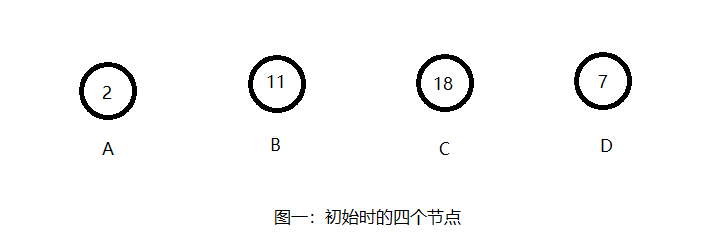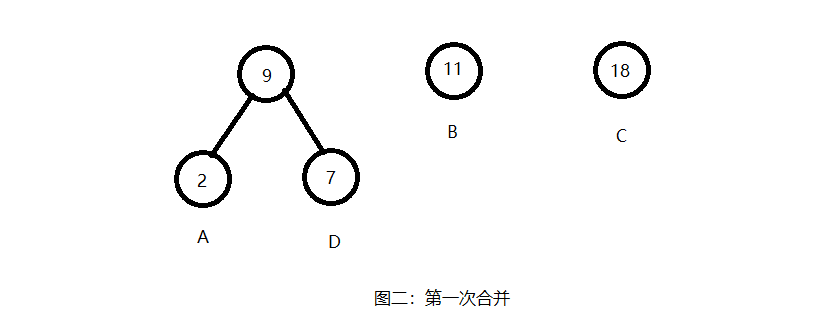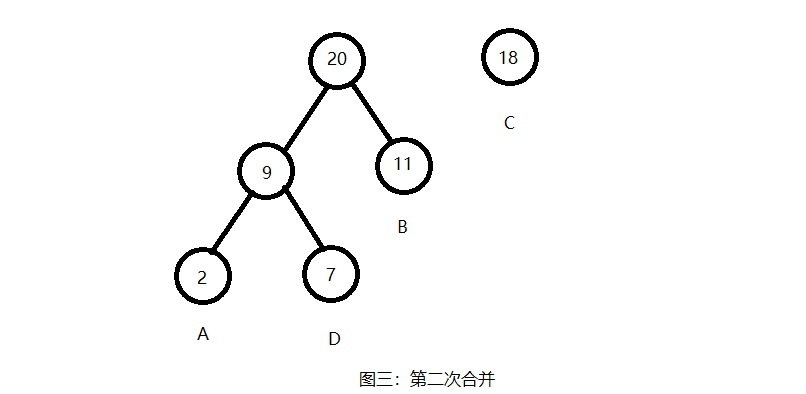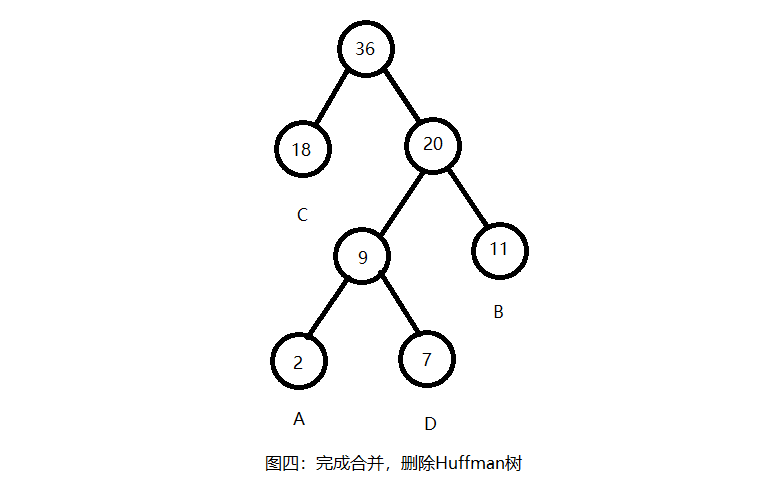如上图所示，经过三次合并之后，我们将的到对应的Huffman树。注意一个小的细节，我们左子树会是权重更小的树，这也更符合我们二叉树的习惯。具体代码：/* 构建函数:根据权重数组构建哈夫曼树
* 参数:无
* 返回值:无
*/
void Huffman::BuildTree() {
// 储存配对堆
PairHeap<Node> ph;

// 将所有的字母生成对于的节点存入配对堆中
for(int c = 0; c < R; c++)
if (freq[c] > 0) {
Node *NewNode = new Node();
NewNode->ch = c;
NewNode->Left = NewNode->Right = NULL;
NewNode->weigth = freq[c];
ph.Insert(*NewNode);
}

// 将配对堆中最小的两个节点取出，进行合并，将合并后的节点重新加入配对堆
// 特别注意:
//		对于Node，应该处理其拷贝构造函数，因为我们将对其进行深拷贝
while (ph.size() > 1) {
Node *x = &ph.Top();
ph.DeleteMin();
Node *y = &ph.Top();
ph.DeleteMin();

Node *Parent = new Node();
Parent->ch = '\0';
Parent->Left = x;
Parent->Right = y;
Parent->weigth = x->weigth + y->weigth;
ph.Insert(*Parent);
}

// 储存根节点同时特殊处理，防止因数据析构而消失
Root = new Node();
*Root = ph.Top();
ph.DeleteMin();
}PS：代码中的PairHeap是我自己写的一个配对堆，代码在这次的blog中并没有贴出来，如果有需要可以给我留言；或者我会在以后的blog中写出来；当然也可以使用其他的优先队列，当然可能会有所不同，具体在Huffman树的Node节点重载需要重新写一下。编码：在我们完成了Huffman树的构建后，我们可以开始构建我们的编码表。这里有个规则：从树根开始，右子树编码为1，左子树编码为0。那么对于图四我们将有如下的编码：A(100)，B(11)，C(0)，D(101)；那么我们如何获得这些编码呢，一个简单的方法是对树进行遍历，同时记录当前的遍历路径，当我们检索到叶子节点的时候根据其存储字符以及路径编写其对应的编码。代码很简单，具体如下：/* 编码函数:根据哈夫曼树构建编码表
* 参数:无
* 返回值:无
*/
void Huffman::BuildCode() {
if(Root != NULL)
BuildCode(Root, "");
}

/* 编码函数:根据对应节点信息进行编码
* 参数:T:当前的编码节点，s:当前的编码信息
* 返回值:无
*/
void Huffman::BuildCode(Node *T, string s) {
// 递归终止条件
if (IsLeaf(T)) {
st[T->ch] = s;
return;
}

// 递归的对下一层节点进行编码
BuildCode(T->Left, s + '0');
BuildCode(T->Right, s + '1');
}展开和压缩：当我们有了Huffman树和编码表后，我们即可进行展开和压缩操作。压缩我们将根据Huffman树进行，当我们读入一个压缩文本的时候，我们将从树根处开始遍历，若读入'0'我们将遍历其左子树，读入'1'遍历其右子树，同时读入文本的下一位。若当前处理的节点为叶子节点，那么我们就会暂时储存其存储的字符，同时也将压缩文本读入下一位，直到压缩文本读完，以此输出所有储存字符。展开操作将根据编码表进行，这将比压缩更加简单。首先是我们需要有其所对应的编码表，之后我们将从原始文本的开头开始读入字符，然后再编码表中找到对应的字符，同时存储其对应的编码。直到所有的字符读入，最后输出我们的压缩编码。具体代码如下：/* 展开函数:根据哈夫曼树展开压缩文本
* 参数:txt:想要进行展开的压缩文本
* 返回值:string:压缩文本展开后的字符串
*/
string Huffman::Expend(string txt) {
// 储存展开结果
string re;

// 遍历整个目标文本
for (int i = 0; i < txt.length(); ) {
if (txt[i] == '#')
break;

Node *x = Root;

// 获取压缩信息
while (!IsLeaf(x)) {
if (txt[i] == '0')
x = x->Left;
else
x = x->Right;
i++;
}

// 更新结果文本
re += x->ch;
}

return re;
}

/* 压缩函数:根据编码表压缩原始文本
* 参数:txt:想要进行压缩的原始文本
* 返回值:string:原始文本压缩后的字符串
*/
string Huffman::Compress(string txt) {
// 储存压缩结果
string re;

// 遍历原始文本同时读取编码表
for (int i = 0; i < txt.length(); i++)
re += st[txt[i]];

return re;
}那么这次的Huffman树到这里就差不多结束了，之后我会补全其完整代码。转载请说明出处哦~~参考文献：《算法——第四版》
展开全文数据压缩
• 一、哈夫曼树的介绍 Huffman Tree，中文名是哈夫曼树或霍夫曼树，它是最优二叉树。 定义：给定n个权值作为n个叶子结点，构造一棵二叉树，若树的带权路径长度达到最小，则这棵树被称为哈夫曼树。 这个定义里面涉及到...
﻿﻿
一、哈夫曼树的介绍
Huffman Tree，中文名是哈夫曼树或霍夫曼树，它是最优二叉树。
定义：给定n个权值作为n个叶子结点，构造一棵二叉树，若树的带权路径长度达到最小，则这棵树被称为哈夫曼树。 这个定义里面涉及到了几个陌生的概念，下面就是一颗哈夫曼树，我们来看图解答。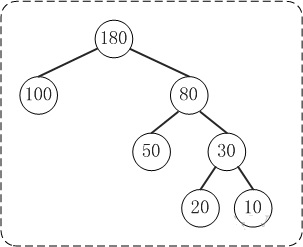(1) 路径和路径长度
定义：在一棵树中，从一个结点往下可以达到的孩子或孙子结点之间的通路，称为路径。通路中分支的数目称为路径长度。若规定根结点的层数为1，则从根结点到第L层结点的路径长度为L-1。
例子：100和80的路径长度是1，50和30的路径长度是2，20和10的路径长度是3。
(2) 结点的权及带权路径长度
定义：若将树中结点赋给一个有着某种含义的数值，则这个数值称为该结点的权。结点的带权路径长度为：从根结点到该结点之间的路径长度与该结点的权的乘积。
例子：节点20的路径长度是3，它的带权路径长度= 路径长度 * 权 = 3 * 20 = 60。
(3) 树的带权路径长度
定义：树的带权路径长度规定为所有叶子结点的带权路径长度之和，记为WPL。
例子：示例中，树的WPL= 1*100 + 2*50 + 3*20 + 3*10 = 100 + 100 + 60 + 30 = 290。
比较下面两棵树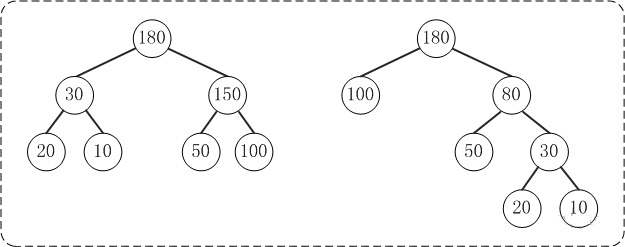上面的两棵树都是以{10, 20, 50, 100}为叶子节点的树。
左边的树WPL=2*10 + 2*20 + 2*50 + 2*100 = 360
右边的树WPL=290
左边的树WPL > 右边的树的WPL。你也可以计算除上面两种示例之外的情况，但实际上右边的树就是{10,20,50,100}对应的哈夫曼树。至此，应该对哈夫曼树的概念有了一定的了解了，下面看看如何去构造一棵哈夫曼树。
二、哈夫曼树的图文解析
假设有n个权值，则构造出的哈夫曼树有n个叶子结点。 n个权值分别设为 w1、w2、…、wn，哈夫曼树的构造规则为：
1. 将w1、w2、…，wn看成是有n 棵树的森林(每棵树仅有一个结点)；
2. 在森林中选出根结点的权值最小的两棵树进行合并，作为一棵新树的左、右子树，且新树的根结点权值为其左、右子树根结点权值之和；
3. 从森林中删除选取的两棵树，并将新树加入森林；
4. 重复(02)、(03)步，直到森林中只剩一棵树为止，该树即为所求得的哈夫曼树。
以{5,6,7,8,15}为例，来构造一棵哈夫曼树。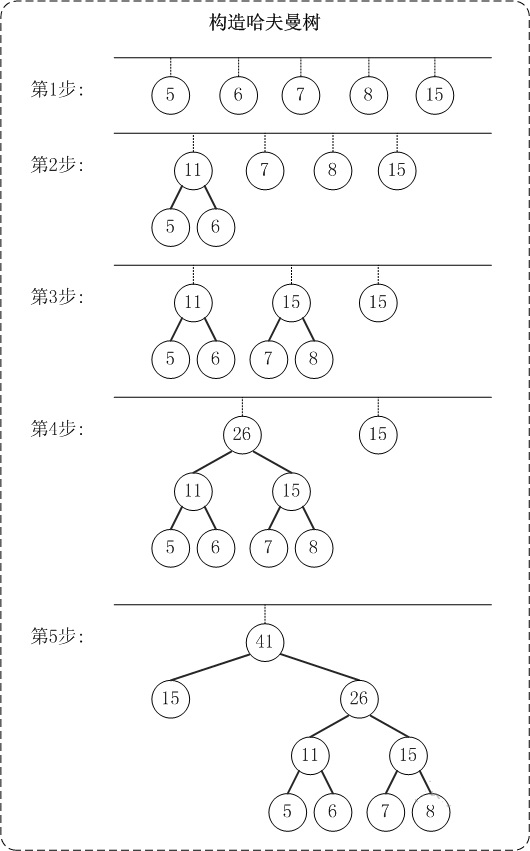第1步：创建森林，森林包括5棵树，这5棵树的权值分别是5,6,7,8,15。
第2步：在森林中，选择根节点权值最小的两棵树(5和6)来进行合并，将它们作为一颗新树的左右孩子(谁左谁右无关紧要，这里，我们选择较小的作为左孩子)，并且新树的权值是左右孩子的权值之和。即，新树的权值是11。 然后，将"树5"和"树6"从森林中删除，并将新的树(树11)添加到森林中。
第3步：在森林中，选择根节点权值最小的两棵树(7和8)来进行合并。得到的新树的权值是15。 然后，将"树7"和"树8"从森林中删除，并将新的树(树15)添加到森林中。
第4步：在森林中，选择根节点权值最小的两棵树(11和15)来进行合并。得到的新树的权值是26。 然后，将"树11"和"树15"从森林中删除，并将新的树(树26)添加到森林中。
第5步：在森林中，选择根节点权值最小的两棵树(15和26)来进行合并。得到的新树的权值是41。 然后，将"树15"和"树26"从森林中删除，并将新的树(树41)添加到森林中。
此时，森林中只有一棵树(树41)。这棵树就是我们需要的哈夫曼树！
三、哈夫曼树的基本操作
哈夫曼树的重点是如何构造哈夫曼树。本文构造哈夫曼时，用到了以前介绍过的"(二叉堆)最小堆"。下面对哈夫曼树进行讲解。
1. 基本定义
public class HuffmanNode implements Comparable, Cloneable {
protected int key;              // 权值
protected HuffmanNode left;     // 左孩子
protected HuffmanNode right;    // 右孩子
protected HuffmanNode parent;   // 父结点

protected HuffmanNode(int key, HuffmanNode left, HuffmanNode right, HuffmanNode parent) {
this.key = key;
this.left = left;
this.right = right;
this.parent = parent;
}

@Override
public Object clone() {
Object obj=null;

try {
obj = (HuffmanNode)super.clone();//Object 中的clone()识别出你要复制的是哪一个对象。
} catch(CloneNotSupportedException e) {
System.out.println(e.toString());
}

return obj;
}

@Override
public int compareTo(Object obj) {
return this.key - ((HuffmanNode)obj).key;
}
}
HuffmanNode是哈夫曼树的节点类。
public class Huffman {

private HuffmanNode mRoot;  // 根结点

...
}
Huffman是哈夫曼树对应的类，它包含了哈夫曼树的根节点和哈夫曼树的相关操作。
2. 构造哈夫曼树
/*
* 创建Huffman树
*
* @param 权值数组
*/
public Huffman(int a[]) {
HuffmanNode parent = null;
MinHeap heap;

// 建立数组a对应的最小堆
heap = new MinHeap(a);

for(int i=0; i<a.length-1; i++) {
HuffmanNode left = heap.dumpFromMinimum();  // 最小节点是左孩子
HuffmanNode right = heap.dumpFromMinimum(); // 其次才是右孩子

// 新建parent节点，左右孩子分别是left/right；
// parent的大小是左右孩子之和
parent = new HuffmanNode(left.key+right.key, left, right, null);
left.parent = parent;
right.parent = parent;

// 将parent节点数据拷贝到"最小堆"中
heap.insert(parent);
}

mRoot = parent;

// 销毁最小堆
heap.destroy();
}
首先创建最小堆，然后进入for循环。
每次循环时：
(1) 首先，将最小堆中的最小节点拷贝一份并赋值给left，然后重塑最小堆(将最小节点和后面的节点交换位置，接着将"交换位置后的最小节点"之前的全部元素重新构造成最小堆)；
(2) 接着，再将最小堆中的最小节点拷贝一份并将其赋值right，然后再次重塑最小堆；
(3) 然后，新建节点parent，并将它作为left和right的父节点；
(4) 接着，将parent的数据复制给最小堆中的指定节点。
四、哈夫曼树的完整源码
1. 哈夫曼树的节点类(HuffmanNode.java)
package com.struction.source.tree.huffman.java;

/**
* Huffman节点类(Huffman.java的辅助类)
*
* @author Anndy
*/

public class HuffmanNode implements Comparable, Cloneable {
protected int key; // 权值
protected HuffmanNode left; // 左孩子
protected HuffmanNode right; // 右孩子
protected HuffmanNode parent; // 父结点

protected HuffmanNode(int key, HuffmanNode left, HuffmanNode right,
HuffmanNode parent) {
this.key = key;
this.left = left;
this.right = right;
this.parent = parent;
}

@Override
public Object clone() {
Object obj = null;

try {
obj = (HuffmanNode) super.clone();// Object 中的clone()识别出你要复制的是哪一个对象。
} catch (CloneNotSupportedException e) {
System.out.println(e.toString());
}

return obj;
}

@Override
public int compareTo(Object obj) {
return this.key - ((HuffmanNode) obj).key;
}
}

2. 哈夫曼树的实现文件(Huffman.java)
package com.struction.source.tree.huffman.java;

/**
* Huffman树
*
* @author Anndy
*/

public class Huffman {

private HuffmanNode mRoot; // 根结点

/*
* 创建Huffman树
*
* @param 权值数组
*/
public Huffman(int a[]) {
HuffmanNode parent = null;
MinHeap heap;

// 建立数组a对应的最小堆
heap = new MinHeap(a);

for (int i = 0; i < a.length - 1; i++) {
HuffmanNode left = heap.dumpFromMinimum(); // 最小节点是左孩子
HuffmanNode right = heap.dumpFromMinimum(); // 其次才是右孩子

// 新建parent节点，左右孩子分别是left/right；
// parent的大小是左右孩子之和
parent = new HuffmanNode(left.key + right.key, left, right, null);
left.parent = parent;
right.parent = parent;

// 将parent节点数据拷贝到"最小堆"中
heap.insert(parent);
}

mRoot = parent;

// 销毁最小堆
heap.destroy();
}

/*
* 前序遍历"Huffman树"
*/
private void preOrder(HuffmanNode tree) {
if (tree != null) {
System.out.print(tree.key + " ");
preOrder(tree.left);
preOrder(tree.right);
}
}

public void preOrder() {
preOrder(mRoot);
}

/*
* 中序遍历"Huffman树"
*/
private void inOrder(HuffmanNode tree) {
if (tree != null) {
inOrder(tree.left);
System.out.print(tree.key + " ");
inOrder(tree.right);
}
}

public void inOrder() {
inOrder(mRoot);
}

/*
* 后序遍历"Huffman树"
*/
private void postOrder(HuffmanNode tree) {
if (tree != null) {
postOrder(tree.left);
postOrder(tree.right);
System.out.print(tree.key + " ");
}
}

public void postOrder() {
postOrder(mRoot);
}

/*
* 销毁Huffman树
*/
private void destroy(HuffmanNode tree) {
if (tree == null)
return;

if (tree.left != null)
destroy(tree.left);
if (tree.right != null)
destroy(tree.right);

tree = null;
}

public void destroy() {
destroy(mRoot);
mRoot = null;
}

/*
* 打印"Huffman树"
*
* key -- 节点的键值 direction -- 0，表示该节点是根节点; -1，表示该节点是它的父结点的左孩子;
* 1，表示该节点是它的父结点的右孩子。
*/
private void print(HuffmanNode tree, int key, int direction) {

if (tree != null) {

if (direction == 0) // tree是根节点
System.out.printf("%2d is root\n", tree.key);
else
// tree是分支节点
System.out.printf("%2d is %2d's %6s child\n", tree.key, key,
direction == 1 ? "right" : "left");

print(tree.left, tree.key, -1);
print(tree.right, tree.key, 1);
}
}

public void print() {
if (mRoot != null)
print(mRoot, mRoot.key, 0);
}
}

3. 哈夫曼树对应的最小堆(MinHeap.java)
package com.struction.source.tree.huffman.java;

/**
* 最小堆(Huffman.java的辅助类)
*
* @author Anndy
*/

import java.util.ArrayList;
import java.util.List;

public class MinHeap {

private List<HuffmanNode> mHeap; // 存放堆的数组

/*
* 创建最小堆
*
* 参数说明： a -- 数据所在的数组
*/
protected MinHeap(int a[]) {
mHeap = new ArrayList<HuffmanNode>();
// 初始化数组
for (int i = 0; i < a.length; i++) {
HuffmanNode node = new HuffmanNode(a[i], null, null, null);
}

// 从(size/2-1) --> 0逐次遍历。遍历之后，得到的数组实际上是一个最小堆。
for (int i = a.length / 2 - 1; i >= 0; i--)
filterdown(i, a.length - 1);
}

/*
* 最小堆的向下调整算法
*
* 注：数组实现的堆中，第N个节点的左孩子的索引值是(2N+1)，右孩子的索引是(2N+2)。
*
* 参数说明： start -- 被下调节点的起始位置(一般为0，表示从第1个开始) end -- 截至范围(一般为数组中最后一个元素的索引)
*/
protected void filterdown(int start, int end) {
int c = start; // 当前(current)节点的位置
int l = 2 * c + 1; // 左(left)孩子的位置
HuffmanNode tmp = mHeap.get(c); // 当前(current)节点

while (l <= end) {
// "l"是左孩子，"l+1"是右孩子
if (l < end && (mHeap.get(l).compareTo(mHeap.get(l + 1)) > 0))
l++; // 左右两孩子中选择较小者，即mHeap[l+1]

int cmp = tmp.compareTo(mHeap.get(l));
if (cmp <= 0)
break; // 调整结束
else {
mHeap.set(c, mHeap.get(l));
c = l;
l = 2 * l + 1;
}
}
mHeap.set(c, tmp);
}

/*
* 最小堆的向上调整算法(从start开始向上直到0，调整堆)
*
* 注：数组实现的堆中，第N个节点的左孩子的索引值是(2N+1)，右孩子的索引是(2N+2)。
*
* 参数说明： start -- 被上调节点的起始位置(一般为数组中最后一个元素的索引)
*/
protected void filterup(int start) {
int c = start; // 当前节点(current)的位置
int p = (c - 1) / 2; // 父(parent)结点的位置
HuffmanNode tmp = mHeap.get(c); // 当前(current)节点

while (c > 0) {
int cmp = mHeap.get(p).compareTo(tmp);
if (cmp <= 0)
break;
else {
mHeap.set(c, mHeap.get(p));
c = p;
p = (p - 1) / 2;
}
}
mHeap.set(c, tmp);
}

/*
* 将node插入到二叉堆中
*/
protected void insert(HuffmanNode node) {
int size = mHeap.size();

filterup(size); // 向上调整堆
}

/*
* 交换两个HuffmanNode节点的全部数据
*/
private void swapNode(int i, int j) {
HuffmanNode tmp = mHeap.get(i);
mHeap.set(i, mHeap.get(j));
mHeap.set(j, tmp);
}

/*
* 新建一个节点，并将最小堆中最小节点的数据复制给该节点。 然后除最小节点之外的数据重新构造成最小堆。
*
* 返回值： 失败返回null。
*/
protected HuffmanNode dumpFromMinimum() {
int size = mHeap.size();

// 如果"堆"已空，则返回
if (size == 0)
return null;

// 将"最小节点"克隆一份，将克隆得到的对象赋值给node
HuffmanNode node = (HuffmanNode) mHeap.get(0).clone();

// 交换"最小节点"和"最后一个节点"
mHeap.set(0, mHeap.get(size - 1));
// 删除最后的元素
mHeap.remove(size - 1);

if (mHeap.size() > 1)
filterdown(0, mHeap.size() - 1);

return node;
}

// 销毁最小堆
protected void destroy() {
mHeap.clear();
mHeap = null;
}
}

4. 哈夫曼树的测试程序(HuffmanTest.java)
package com.struction.source.tree.huffman.java;

/**
* Huffman树的测试程序
*
* @author Anndy
*/

public class HuffmanTest {

private static final int a[] = { 5, 6, 8, 7, 15 };

public static void main(String[] args) {
int i;
Huffman tree;

System.out.print("== 添加数组: ");
for (i = 0; i < a.length; i++)
System.out.print(a[i] + " ");

// 创建数组a对应的Huffman树
tree = new Huffman(a);

System.out.print("\n== 前序遍历: ");
tree.preOrder();

System.out.print("\n== 中序遍历: ");
tree.inOrder();

System.out.print("\n== 后序遍历: ");
tree.postOrder();
System.out.println();

System.out.println("== 树的详细信息: ");
tree.print();

// 销毁二叉树
tree.destroy();
}
}

展开全文• 实验报告 实验名称 哈弗曼编码和译码 专业班级 电子信息科学与技术0904 姓 名 秦杰 学 号 1404090506 指导教师 胡志坤 2011年 11月 实验四哈夫曼编码和译码 实验目的和要求 掌握哈夫曼树的基本概念及其存储结构 ...
• ## 数据结构18————哈夫曼树

千次阅读 多人点赞 2017-12-21 22:10:51
数据结构15————哈夫曼树 一.内容 1.哈夫曼树的定义和原理 2.哈夫曼树的建立 3.哈夫曼编码 4.哈夫曼算法的实现
数据结构18————哈夫曼树
文章目录数据结构18————哈夫曼树一.内容二.哈夫曼树的定义和原理1.哈夫曼树的定义及作用2.哈夫曼树的相关概念三.哈夫曼树的建立1.思想2.例子3.如何存储4.静态三叉链表实现二叉树的存储四.哈夫曼编码1.编码2.哈夫曼编码3.例子五.哈夫曼算法1.结构体的设计2.哈夫曼树的建立3.输出所有字符的编码4.编码字符串5.译码6.带权路径长(WPL)六.源代码七.参考资料
一.内容
哈夫曼树的定义和原理
哈夫曼树的建立
哈夫曼编码
哈夫曼算法的实现
二.哈夫曼树的定义和原理
1.哈夫曼树的定义及作用

哈夫曼树的又称为最优二叉树,是带权路径最短的树，可用来构造最优编码，常用于数据压缩和信息传递

2.哈夫曼树的相关概念
路径:从一个结点到另一个结点之间的分支路径
路径长度:从一个结点到另一结点所经过的分支数目
结点的权值:树中每个结点所赋予的具有某种实际意义的实数
带权路径长度:从树根到某一结点的路径长度与该结点的权的乘积。
树的带权路径长度(WPL):树中从根到所有叶子结点的各个带权路径长度之和。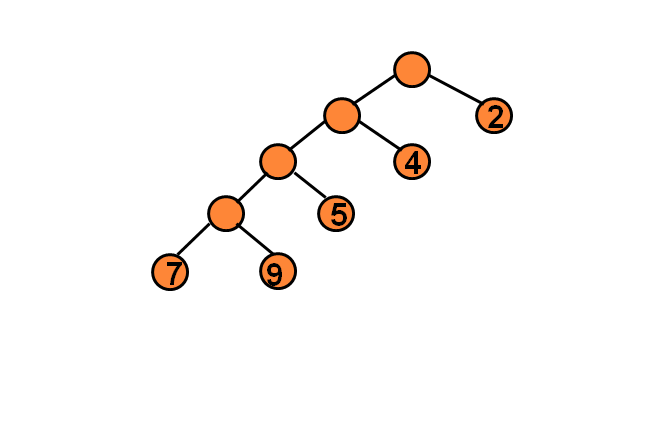如图所示的树,其WPL=7x4+9x4+5x3+4x2+2x1=89
所以哈夫曼树就是,相同叶子结点所构成的树中，WPL最小的一颗。
三.哈夫曼树的建立
如果给定叶子节点的权值，如何构造出一颗WPL最小的树呢？这个过程就是构造哈夫曼树的过程
1.思想
每次选择节点集合中最小的两个节点，构成一颗树,根节点的权值为两个之和，将根节点加入节点集合，重复上述过程。
形式化表述

1.根据给定的n个权值{w1,w2……wn},构造出n颗二叉树的集合F={T1.T2……Tn},其中每一棵二叉树均只含一个带权值为wi的根节点，其左右子树为空树
2.在F中选择其根节点为权值最小的两颗二叉树，分别作为左右子树构造出一颗新的二叉树。并置这颗新树根节点权值为左右根节点的权值之和。
3.在F中删去这两棵树，同时加入新生成的树
4.重复23过程，直到F中只剩一颗树为止。

2.例子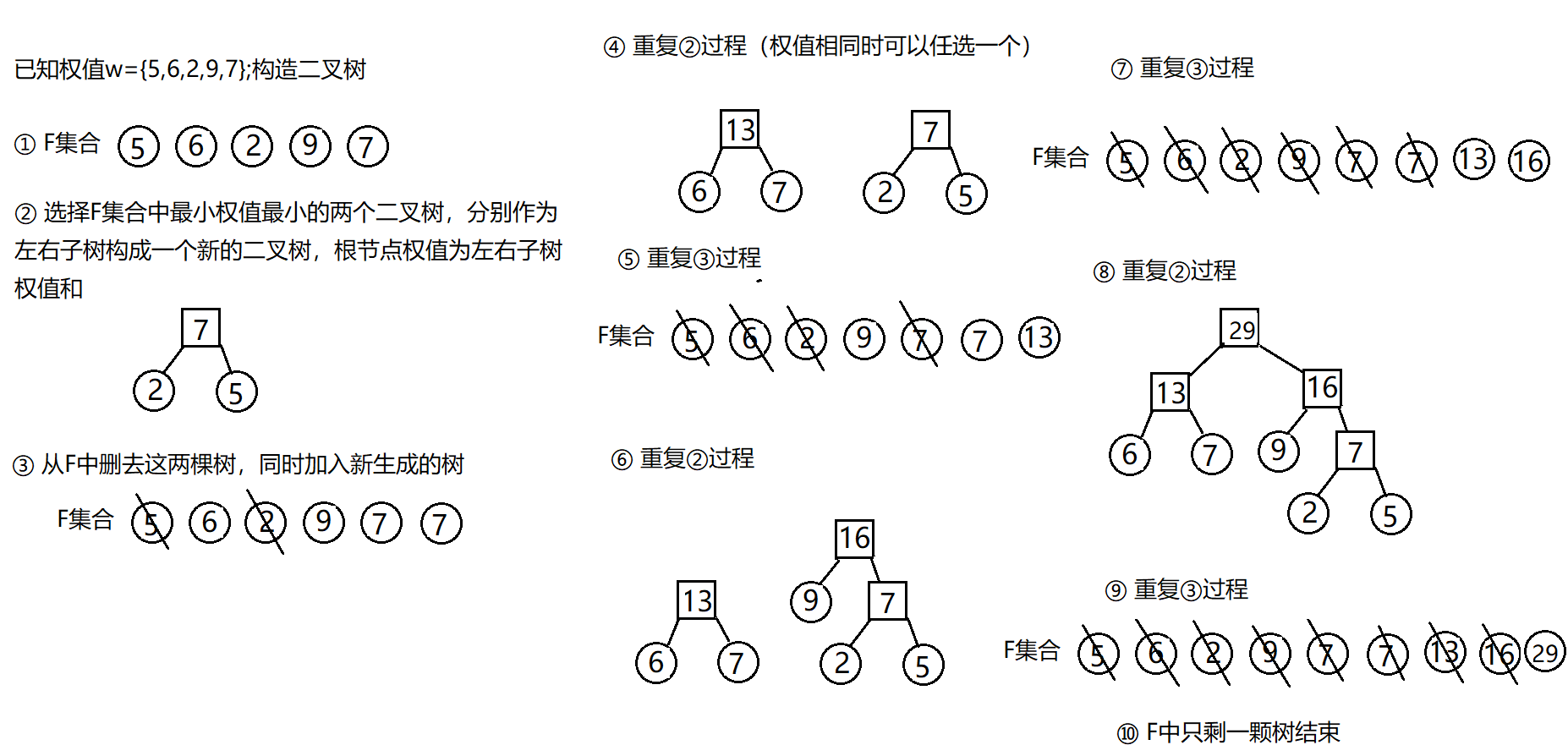3.如何存储
哈夫曼树的存储可以使用三叉链表和静态三叉链表，为了简单考虑，一般选择静态三叉链表来实现哈夫曼树的存储
4.静态三叉链表实现二叉树的存储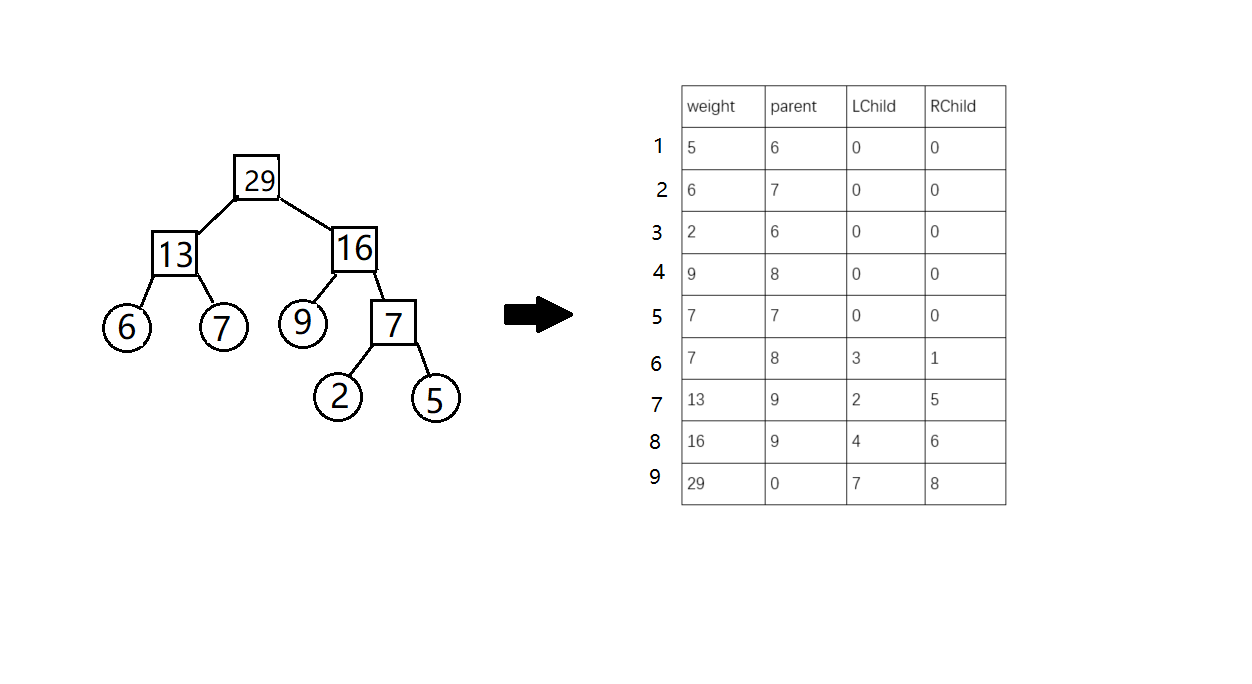四.哈夫曼编码
我们前文说过，哈夫曼树主要应用是在数据压缩信息传递中，而如何应用哈夫曼树进行数据的压缩，这就涉及到哈夫曼树编码。
1.编码
编码是什么?
举个例子如果我们需要通过网络传输一段文字；ABCDEFGH，那么很容易想到，让A=000，B=001,C=010,D=011,F=100,G=101,H=111。所以ABCDEFG就可以表示为000.001.010.011.100.101.110.111。通过这个规则，就可以表示ABCDEFG所构成的任意一段文字。文字转换出的二进制就是编码。
上面的编码可以解决大部分的问题，但是否可以进行更好的优化，比如让它编码后的二进制长度尽可能的达到更小。
如何达到呢？我们可以通过让出现频率大的编码尽可能的短，出现概率小的编码啊可以适当的长点。但是存在一个问题，比如说AB出现的频率大，让A=0，B=1，C出现的频率小，让C=01。那么问题来了，01代表AB还是代表C。所以如果要设计长度不等的编码必须满足

任何一个字符编码都不是另一个字符的编码的前缀，这种编码就是前缀编码

2.哈夫曼编码
哈夫曼编码就是一种前缀编码,而且是编码后最短的前缀编码。
哈夫曼树的构建

将每个字符出现的次数作为权值，按照哈夫曼树的构建过程组成的一颗二叉树

哈夫曼编码

从哈夫曼树的根节点出发，左子树输出0，右子树输出1。知道达到叶子节点后停止，此时输出的二进制就是该叶子节点的哈夫曼编码

3.例子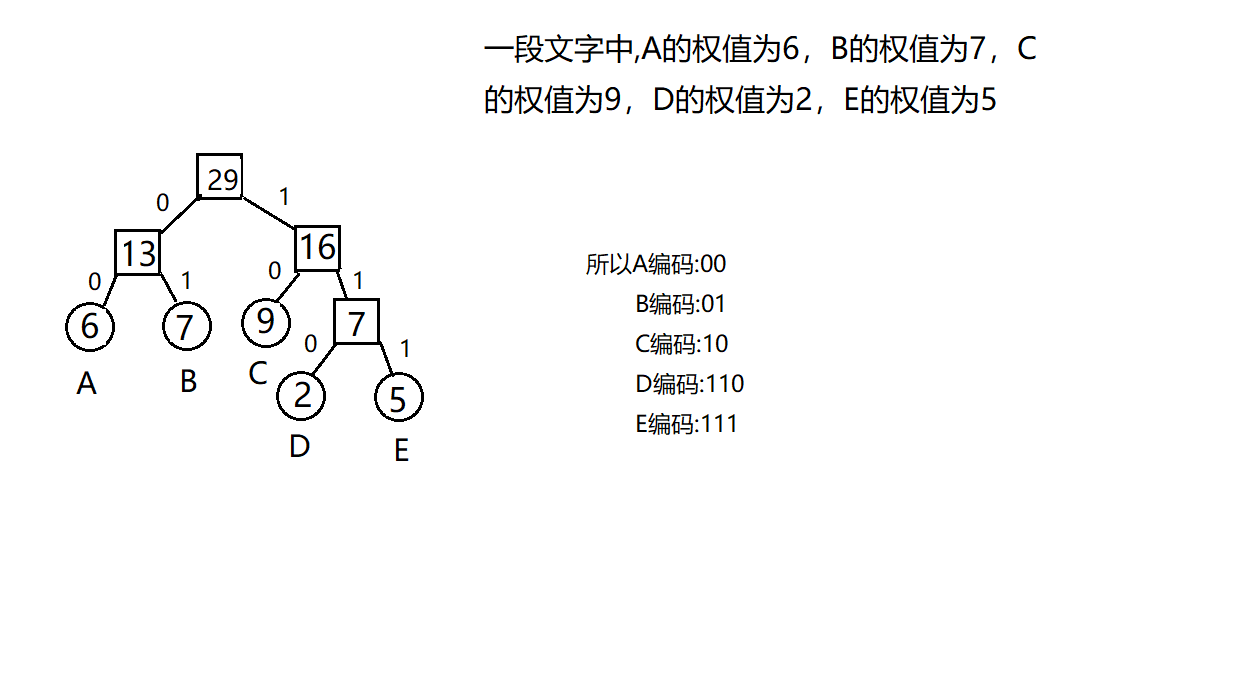五.哈夫曼算法
我的代码设计未舍弃下标为0的位置，所以叶子节点从0开始
1.结构体的设计
#include <stdio.h>
#define N 30
#define M 2*N-1
typedef struct{
int weight;
char data;
int parent,Lchild,Rchild;
}HTNode,HuffmanTree[M+1];

2.哈夫曼树的建立
void CrtHuffmanTree(HuffmanTree	ht,int n){ //创建哈夫曼树
int m=2*n-1;
int i;
int s1,s2;
for(i=0;i<n;i++){       //初始化叶子节点
scanf("%d",&ht[i].weight);
ht[i].data = 'A'+i;
ht[i].parent=-1;
ht[i].Lchild=-1;
ht[i].Rchild=-1;
}
for(i=n;i<m;i++){   //初始化非叶子节点
ht[i].weight=0;
ht[i].parent=-1;
ht[i].Lchild=-1;
ht[i].Rchild=-1;
}

for(i=n;i<m;i++) //创建哈夫曼树
{
seek(ht,i,&s1,&s2);	 //寻找ht中i之前最小的两个元素下标，s1<s2;
ht[s1].parent=i;
ht[s2].parent=i;
ht[i].weight = ht[s1].weight+ht[s2].weight;
ht[i].Lchild = s1;
ht[i].Rchild = s2;
}

}

void seek(HuffmanTree ht,int n,int *s1,int *s2){  //寻找ht中i之前最小的两个元素下标，s1<s2;
int i,j;
for(i=0;i<n&&ht[i].parent!=-1;i++); //s1,s2的初始化
j=i;
i++;
for(;i<n&&ht[i].parent!=-1;i++);
*s1 = ht[i].weight<ht[j].weight?i:j;
*s2 = ht[i].weight<ht[j].weight?j:i;
i++;
while(i<n){
if(ht[i].parent!=-1){

}else if(ht[i].weight<ht[*s1].weight){
*s2 = *s1;
*s1 = i;
}else if(ht[i].weight<ht[*s2].weight){
*s2 = i;

}
i++;
}
}

3.输出所有字符的编码
void codinghuffman(HuffmanTree	ht,int n){ //编码所有的叶子节点
int i,j;
char str[N];
int p,q; //p当前节点，q是p的双亲节点
for(i=0;i<n;i++){
p=i;
for(j=0;ht[p].parent!=-1;j++){

q=ht[p].parent;
if(p==ht[q].Lchild){ //如果是左节点,则为0
str[j]='0';
}else{           //如果是右节点,则为1
str[j]='1';
}
p=ht[p].parent;
}

printf("%c:",ht[i].data); //打印结果
for(j=j-1;j>=0;j--){
printf("%c",str[j],j);
}
printf("\n");
}
}

4.编码字符串
void aCodinghuffman(HuffmanTree	ht){ //编码字符串
int i,j;
char in[N];
char str[N];
int p,q;
getchar();
gets(in);
for(i=0;in[i];i++){ //遍历每个字母
p=in[i]-'A'; //确定该叶子节点存储的位置,这句根据具体问题而定
for(j=0;ht[p].parent!=-1;j++){    //解码每个字母

q=ht[p].parent;
if(p==ht[q].Lchild){ //如果是左孩子
str[j]='0';
}else{            //如果是右孩子
str[j]='1';
}
p=ht[p].parent;
}
for(j=j-1;j>=0;j--){  //打印结果
printf("%c",str[j],j);
}
}
printf("\n");
}

5.译码
void decodeHuffmanTree(HuffmanTree ht,int n){ //译码
int i,j;
char str[N];
int p=2*n-2; // p当前节点，p当前节点儿子

gets(str);
for(i=0;str[i];i++){
if(str[i]=='1'){
p = ht[p].Rchild;
}else if(str[i]=='0'){
p = ht[p].Lchild;
}
if(ht[p].Lchild==-1&&ht[p].Rchild==-1){  //当前字段解码完毕
printf("%c",ht[p].data);
p =2*n-2;
}
}
}

6.带权路径长(WPL)
int WPL(HuffmanTree ht,int n){
int wpl=0;
int h;
int p;
int i;
for(i=0;i<n;i++){
h=0;
for(p=i;ht[p].parent!=-1;p=ht[p].parent){
h++;
}
wpl = wpl+ h*ht[i].weight;
}
return wpl;
}

六.源代码
text15中
七.参考资料
《大话数据结构》
《数据结构与算法分析》


展开全文数据压缩
• 哈夫曼树（最优二叉树） 百度百科：https://baike.baidu.com/item/%E5%93%88%E5%A4%AB%E6%9B%BC%E6%A0%91/2305769?fr=aladdin 一. 目的： 找出存放一串字符所需的最少的二进制编码 二. 构造方法： 首先统计出每种...
• 文章目录哈夫曼树和哈夫曼编码哈夫曼树引子定义与原理 哈夫曼（Huffman）编码是个实用的压缩编码方案。 哈夫曼树 引子 我们观察以下代码： if( a < 60) { printf("不及格\n"); }else if( a < 70){ printf(...链表 算法
• 1.哈夫曼树：给定n个权值作为n个叶子结点，构造一棵二叉树，若带权路径长度达到最小，称这样的二叉树为最优二叉树，也称为哈夫曼树(Huffman Tree)。哈夫曼树共有2*n-1个结点（性质）。 2.哈夫曼树构建：选取权值...哈夫曼编码 哈夫曼译码
• //哈夫曼树结点结构 typedef struct { int weight;//结点权重 int parent, left, right;//父结点、左孩子、右孩子在数组中的位置下标 }HTNode, *HuffmanTree; //动态二维数组，存储哈夫曼编码 typedef c
• 二、实验内容 1.根据给出的字符以及这些字符的使用频率构建哈夫曼树。 2.根据哈夫曼树对字符进行哈夫曼编码，并保存这些编码。...1. 建立哈夫曼树存储结构和哈夫曼编码的存储结构。 2. 建立哈夫曼树的...
• 假设有n个权值，则构造出的哈夫曼树有n个叶子结点。 n个权值分别设为 w1、w2、…、wn，则哈夫曼树的构造规则为： 　(1) 将w1、w2、…，wn看成是有n 棵树的森林(每棵树仅有一个结点)； 　(2) 在森林中选出两个根结点...c++ 算法
• 二叉树的存储结构哈夫曼树及编码A. 构造哈夫曼树a. 频度统计b. 生成哈夫曼树B. 哈夫曼编码C. 解码 Ⅰ 树 由于树的应用场合很少，不是很实用，所以在此只做简单介绍。 A. 树的概念 树状图是一种数据结...算法 c语言
• 树-树的定义及存储结构、二叉树、遍历二叉树、线索二叉树、哈夫曼树（C语言描述） 此文章仅作为自己学习过程中的记录和总结，同时会有意地去用英文来做笔记，一些术语的英译不太准确，内容如有错漏也请多指教，谢谢...链表
• 大部分代码是以前写的，所以是C语言，只完成了生成哈夫曼树。 复习期间又完善了哈夫曼树生成编码的部分。 如果使用C++/Java实现，利面向对象的优势应该更好。 这里不再阐述哈夫曼编码为何是最短前缀编码的理论知识 ...算法 c语言
• 文章目录1、先掌握几个概念1.1 什么是路径？...  先听一遍哈夫曼树的概念：给定n个权值作为n个叶子结点，构造一棵二叉树，若该树的带权路径长度（WPL）达到最小，称这样的二叉树为最优二叉树，也成为哈夫曼树（Huf...哈夫曼编码 java
• 1、哈夫曼树 1.1哈夫曼树基本介绍 给定n个权值作为n个叶子结点，构造一棵二叉树，若该树的带权路径长度(wpl) 达到最小，称这样的二叉树为最优二叉树，也称为哈夫曼树(Huffman Tree) 路径：在一棵树中，从一个结点往...最优二叉树 哈夫曼编码 文件压缩 文件解压
• 哈夫曼树 1.1基本介绍 给定n个权值作为n个叶子结点，构造一棵二叉树，若该树的带权路径长度(wpl)达到最小，称这样的二叉树为最优二叉树，也称为哈夫曼树(Huffman Tree), 还有的书翻译为霍夫曼树。 赫夫曼树是带权...
• ## 哈夫曼树、哈夫曼编码详解

千次阅读 多人点赞 2021-06-17 09:33:06
哈夫曼树、哈夫曼编码，也就这么一回事，一文搞懂！算法 哈夫曼编码
• 目录八大数据结构——哈夫曼树（七）基础定义哈夫曼树的构造哈夫曼树编码哈夫曼树解码代码实现完整代码 树是数据结构中非常重要的一项，有关树的数据结构有许多种，本文重点研究的是哈夫曼树（最优二叉树）。 基础...java
• 用python实现哈夫曼树与哈夫曼编码，并通过哈夫曼编码压缩核酸序列信息。Python
• ## 哈夫曼树及其应用

千次阅读 2021-02-13 19:26:16
哈夫曼树及其应用 树结构是一种应用非常广泛的结构，在一些特定应用中，树具有一些特殊特点，利用这些特点能解决很多工程问题。下面以哈夫曼树为例，说明二叉树的一个具体应用。 基本概念 哈夫曼树又称最优树，是一...指针 算法 c语言
• 1. 哈夫曼树 哈夫曼树也称作最优二叉树，当树中的节点带了权重信息了，带权路径长度最小的二叉树叫做最优二叉树。带权路径长度=sum（权重*度）。sum代表每个节点的之和。加入有如下带权重的节点。  权重分别是1、...
• 哈夫曼树 首先我们需要明白什么是哈夫曼树： 概念上说，哈夫曼树是给定一组具有确定权值的叶子结点，带权路径长度最小的二叉树。 叶子结点的权值：对叶子结点赋予的一个有意义的数值量。 二叉树的带权路径长度：设...算法 编程语言
• 之前做的一个数据结构作业，通过哈夫曼树实现对文本的压缩与解压，参考了很多网上的方法，因为时间有限，注释并没有写，但是代码缩进还是比较清晰。 另外，哈夫曼树我单独写了个头文件，是在之前写二叉树类的基础上...算法 压缩算法
• 前置：C++数据结构_树的理论学习笔记（2）_存储结构，二叉树的实现 ...哈夫曼树又称最优二叉树,是一种带权路径长度最短的二叉树。树的带权路径长度, 就是树中所有叶结点的权值乘上其到根结点的路径长度(若根结点...c++ 算法
• 哈夫曼树 并查集是一种用来管理元素分组情况的数据结构，可以高效地进行查询、合并操作，但无法进行分割操作。 1.查询元素a和元素b是否属于同一组 2.合并元素a和元素b所在的组 并查集使用树形结构实现，但不是...算法 c++
• ## 【笔记】哈夫曼树

千次阅读 2017-11-01 00:34:54
哈夫曼树的概念 哈夫曼树的构造算法 哈夫曼编码 哈夫曼编码算法的实现哈夫曼编码 哈夫曼算法 最优二叉树
• 哈夫曼树和哈夫曼编码哈夫曼树的定义哈夫曼树的构造哈夫曼编码 哈夫曼树的定义 树的结点常常赋予一个表示某种意义的数值，称为结点的权。从树的根结点到任意结点的路径长度与该结点上权值的乘积，称为该结点的带权...
• 哈夫曼树原理，及构造方法 概述 给定n个权值作为n个叶子结点，构造一棵二叉树，若该树的带权路径长度达到最小，称这样的二叉树为最优二叉树，也称为哈夫曼树(Huffman Tree)。哈夫曼树是带权路径长度最短的树，权值...哈夫曼编码...